https://doi.org/10.5194/acp-21-16067-2021
https://doi.org/10.5194/acp-21-16067-2021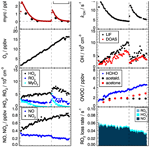# Atmospheric photo-oxidation of myrcene: OH reaction rate constant, gas-phase oxidation products and radical budgets

Zhaofeng Tan, Luisa Hantschke, Martin Kaminski, Ismail-Hakki Acir, Birger Bohn, Changmin Cho, Hans-Peter Dorn, Xin Li, Anna Novelli, Sascha Nehr, Franz Rohrer, Ralf Tillmann, Robert Wegener, Andreas Hofzumahaus, Astrid Kiendler-Scharr, Andreas Wahner, and Hendrik Fuchs
Abstract

The photo-oxidation of myrcene, a monoterpene species emitted by plants, was investigated at atmospheric conditions in the outdoor simulation chamber SAPHIR (Simulation of Atmospheric PHotochemistry In a Large Reaction Chamber). The chemical structure of myrcene consists of one moiety that is a conjugated π system (similar to isoprene) and another moiety that is a triple-substituted olefinic unit (similar to 2-methyl-2-butene). Hydrogen shift reactions of organic peroxy radicals (RO2) formed in the reaction of isoprene with atmospheric OH radicals are known to be of importance for the regeneration of OH. Structure–activity relationships (SARs) suggest that similar hydrogen shift reactions like in isoprene may apply to the isoprenyl part of RO2 radicals formed during the OH oxidation of myrcene. In addition, SAR predicts further isomerization reactions that would be competitive with bimolecular RO2 reactions for chemical conditions that are typical for forested environments with low concentrations of nitric oxide. Assuming that OH peroxy radicals can rapidly interconvert by addition and elimination of O2 like in isoprene, bulk isomerization rate constants of 0.21 and 0.097 s−1 (T=298K) for the three isomers resulting from the 3-OH and 1-OH addition, respectively, can be derived from SAR. Measurements of radicals and trace gases in the experiments allowed us to calculate radical production and destruction rates, which are expected to be balanced. The largest discrepancies between production and destruction rates were found for RO2. Additional loss of organic peroxy radicals due to isomerization reactions could explain the observed discrepancies. The uncertainty of the total radical (${\mathrm{RO}}_{x}=\mathrm{OH}+{\mathrm{HO}}_{\mathrm{2}}+{\mathrm{RO}}_{\mathrm{2}}$) production rates was high due to the uncertainty in the yield of radicals from myrcene ozonolysis. However, results indicate that radical production can only be balanced if the reaction rate constant of the reaction between hydroperoxy (HO2) and RO2 radicals derived from myrcene is lower (0.9 to $\mathrm{1.6}×{\mathrm{10}}^{-\mathrm{11}}$cm3 s−1) than predicted by SAR. Another explanation of the discrepancies would be that a significant fraction of products (yield: 0.3 to 0.6) from these reactions include OH and HO2 radicals instead of radical-terminating organic peroxides. Experiments also allowed us to determine the yields of organic oxidation products acetone (yield: 0.45±0.08) and formaldehyde (yield: 0.35±0.08). Acetone and formaldehyde are produced from different oxidation pathways, so that yields of these compounds reflect the branching ratios of the initial OH addition to myrcene. Yields determined in the experiments are consistent with branching ratios expected from SAR. The yield of organic nitrate was determined from the gas-phase budget analysis of reactive oxidized nitrogen in the chamber, giving a value of 0.13±0.03. In addition, the reaction rate constant for myrcene +OH was determined from the measured myrcene concentration, yielding a value of $\left(\mathrm{2.3}±\mathrm{0.3}\right)×{\mathrm{10}}^{-\mathrm{10}}$cm3 s−1.

Share
Dates
1 Introduction

Monoterpenes are emitted from vegetation accounting for approximately 160 Tg of the 1000 Tg of biogenic volatile organic compounds (VOCs) that are released into the atmosphere per year . Monoterpenes are highly reactive to the major oxidants in the atmosphere, hydroxyl radicals (OH), ozone (O3), and nitrate radicals (NO3) and thus play an important role in ozone and secondary organic aerosol formation .

Myrcene emissions contribute to the total biogenic monoterpene emissions in the range of 2 % to 10 % . Emission rates highly depend on the type of tree and season of the year . In addition, there are hints for anthropogenic sources from the analysis of the composition of indoor air . Few studies have been conducted to investigate the oxidation of myrcene . In these studies, acetone, formaldehyde and 4-vinyl-pentenal have been identified as major oxidation products from the reaction of myrcene with OH, but yields determined in these studies vary. reported an organic nitrate yield of 10 % from the oxidation of myrcene by direct measurements using mass spectrometry. The reaction rate constant of myrcene +OH was determined to be $\left(\mathrm{2.1}±\mathrm{0.2}\right)×{\mathrm{10}}^{-\mathrm{10}}$ and $\left({\mathrm{3.4}}_{-\mathrm{1.0}}^{+\mathrm{1.5}}\right)×{\mathrm{10}}^{-\mathrm{10}}$ at room temperature, with a discrepancy of up to 60 %. These results demonstrate the photo-oxidation of myrcene requires further investigation.

There is no detailed chemical mechanism of myrcene degradation by OH in the literature. The acyclic structure of myrcene consists of two parts: an isoprenyl part (${\mathrm{CH}}_{\mathrm{2}}=\mathrm{CH}-\mathrm{C}\left(={\mathrm{CH}}_{\mathrm{2}}\right){\mathrm{CH}}_{\mathrm{2}}-$moiety) and another part that is structurally similar to 2-methyl-2-butene ($\left({\mathrm{CH}}_{\mathrm{3}}{\right)}_{\mathrm{2}}\mathrm{C}=\mathrm{CH}-{\mathrm{CH}}_{\mathrm{2}}-$moiety) (Fig. 1). Recent investigations of the oxidation of isoprene revealed that organic peroxy radicals (RO2) formed after the attack of OH to isoprene can rapidly interconvert, so that fast H-atom migration reactions of specific RO2 isomers with initially low yield can significantly gain in importance. These reactions impact atmospheric chemistry most if they become competitive with bimolecular loss reactions such as reactions with nitric oxide (NO) and hydroperoxy radicals (HO2). In the case of isoprene, these isomerization reactions can eventually regenerate OH radicals, so that a high OH regeneration efficiency of 0.5 can be sustained in the atmosphere also in regions where radical termination reactions have been thought to dominate the fate of radicals . Therefore, radical regeneration from isomerization reactions helped partly to explain observations of unexpectedly high OH concentrations in field experiments . Radical regeneration from RO2 isomerization reactions has also been shown to play a role in the oxidation of methacrolein , 3-pentanone , glyoxal , n-hexane and 2-hexanol , hydroxymethyl hydroperoxides , and 2-hydroperoxy-2-methylpentane .

In this study, the oxidation of myrcene by OH was investigated in the atmospheric simulation chamber SAPHIR (Simulation of Atmospheric PHotochemistry In a Large Reaction Chamber) at Forschungszentrum Jülich. Experiments were performed under controlled conditions with concentrations of trace gases and radicals typical for atmospheric conditions. Experiments aim for adding to the determination of a degradation mechanism for myrcene.

2 Oxidation mechanism of myrcene

Myrcene is an acyclic hydrocarbon with three unsaturated carbon double bonds to which OH preferentially adds. One end of the myrcene molecule is structurally similar to isoprene, and the other end is similar to 2-methyl-2-butene (Fig. 1). The myrcene oxidation by OH forms 10 isomers of peroxy radicals (MyO2) (Fig. 2). Structure–activity relationships (SARs) by suggest that OH preferably adds to the double bond of the isolated $-\mathrm{CH}=\mathrm{C}\left({\mathrm{CH}}_{\mathrm{3}}{\right)}_{\mathrm{2}}$ moiety producing two peroxy radical species with yields of 17 % (7-OH-6-OO radical) and 31 % (6-OH-7-OO radical) (Fig. 2). When OH adds to the conjugated carbon double bonds, six isomeric hydroxy peroxy radicals are formed like in the OH or NO3 oxidation of isoprene . Addition to the ${\mathrm{C}}_{{\mathrm{3}}^{\prime }}$ position yields two allyl-like hydroxy radical isomers producing E-3-OH-1-OO, 3-OH-3-OO and Z-3-OH-1-OO peroxy radicals after the subsequent addition of O2. A total yield of 31 % is estimated by SAR (Peeters et al., 2007) for these three isomers. Similarly, OH addition to the C1 position followed by addition of O2 to the allyl radical structure leads to the formation of E-1-OH-3-OO, 1-OH-2-OO and Z-1-OH-3-OO radicals with a total yield of 17 %. In the isoprene mechanism developed by , and , a central element is the fast interconversion reactions between the OH adducts and corresponding OH peroxy radicals that proceed by addition and elimination of O2. Similar reactions are expected for the isoprene structure in myrcene (Fig. 2) establishing a coupled equilibrium between MyO2 isomers.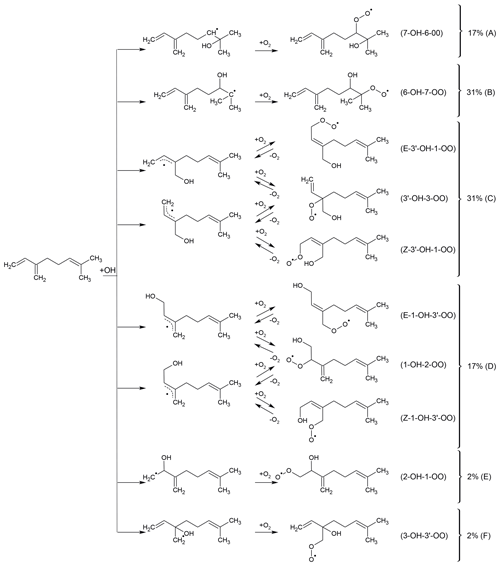Figure 2Peroxy radicals formed from the addition of OH to the double bonds in myrcene. MyO2 formed from the OH addition to the isoprenyl part of myrcene leads to the formation of MyO2 species that are expected to rapidly interconvert by oxygen abstraction and elimination like in isoprene . Assuming reaction rate constants for oxygen abstraction and elimination like in isoprene, ${\mathrm{3}}^{\prime }-\mathrm{OH}-\mathrm{3}-\mathrm{OO}$ and $\mathrm{1}-\mathrm{OH}-\mathrm{2}-\mathrm{OO}$ are expected to have the highest yields among the MyO2 species that can interconvert. MyO2 yields are predicted using SAR by .

For OH attack on the C2 or C3 position of myrcene, the resulting OH adducts are not resonance stabilized and are hence not as favourable as the allylic-type radicals produced from C1 or ${\mathrm{C}}_{{\mathrm{3}}^{\prime }}$ addition. Yields of these MyO2 radicals are expected to be less than 2 %, and therefore the chemistry of these two minor isomers is not further discussed in this work.

All MyO2 isomers can undergo bimolecular reactions with NO, HO2 and other RO2 species. Specific reaction rate constants have not been measured, but values are expected to be within the range of typical rate constants for organic peroxy radicals. For example, SAR by suggests a value of $\mathrm{2.2}×{\mathrm{10}}^{-\mathrm{11}}$cm3 s−1 (T=298K) of the reaction rate constants for the reaction of monoterpene-derived RO2 (including MyO2) with HO2. Reaction products of the reaction of RO2 with HO2 are expected to be organic peroxides.

The reaction of the 6-OH-7-OO and 7-OH-6-OO radicals with NO produces mainly 4-vinyl-4-pentenal, acetone and HO2 (Fig. 3) as shown in experimental studies as well as predicted by SAR . and suggested additional pathways for the alkoxy radical that is formed in the reaction with NO of these two MyO2 species. These pathways would include rearrangement reactions followed by reaction with NO and fragmentation. This was suggested to explain the observation of organic species with various masses ($m/z=\mathrm{71}$, $m/z=\mathrm{113}$, $m/z=\mathrm{115}$) detected by the proton-transfer-reaction mass spectrometry instrument in . Similarly, suggested a reaction pathway of 6-OH-7-OO and 7-OH-6-OO radicals to explain observed terpenylic acid in their experiments. The reaction of the other two most abundant radicals, 1-OH-2-OO and 3-OH-3-OO, with NO would lead to the formation of HO2 and formaldehyde together with 2-methyldiene-6-methyl-5-heptenal and 1-vinyl-5-methyl-4-hexanone, respectively (Fig. 3).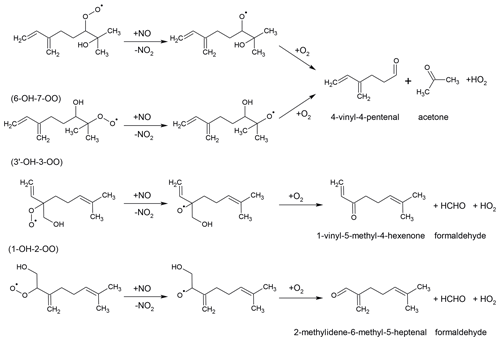Figure 3Reactions of the four most abundant myrcene hydroxy peroxy radicals with NO forming HO2 and carbonyl compounds.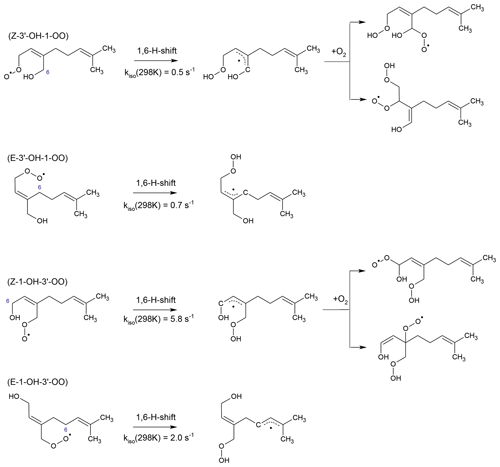Figure 4Fast H-shift reactions of myrcene peroxy radicals. Rate constants apply to 298 K. They are adopted for the $\mathrm{Z}-{\mathrm{3}}^{\prime }-\mathrm{OH}-\mathrm{1}-\mathrm{OO}$ and $\mathrm{Z}-\mathrm{1}-\mathrm{OH}-{\mathrm{3}}^{\prime }-\mathrm{OO}$ isomers from the corresponding 1,6-H-shift reactions of isoprene peroxy radicals . Similar subsequent chemistry like for isoprene leads to the formation of peroxy radicals, some of which rapidly decompose. The isomerization reaction rate constants for the $\mathrm{E}-{\mathrm{3}}^{\prime }-\mathrm{OH}-\mathrm{1}-\mathrm{OO}$ and $\mathrm{E}-\mathrm{1}-\mathrm{OH}-{\mathrm{3}}^{\prime }-\mathrm{OO}$ peroxy radicals are calculated using SAR by . Subsequent chemistry of products from these reactions likely undergoes fast ring-closure reactions on the dimethyl double bond (not shown here).

In addition to bimolecular reactions, unimolecular reactions can be of importance for specific MyO2. Due to the similarity of the conjugated double-bond structure in myrcene and isoprene, it can be expected that H-shift reactions found to be important in isoprene apply for corresponding MyO2 radicals. Therefore, Z-3-OH-1-OO and Z-1-OH-3-OO radicals are expected to undergo α-OH 1,6-H migration followed by O2 addition leading to hydroperoxy peroxy radicals (Fig. 4). As a first approximation, reaction rate constants can be assumed to be on the order of 1 s−1 at 298K like for corresponding reactions in isoprene . Similar products from further isomerization and decomposition reactions could be expected as predicted for isoprene . This would also lead to the regeneration of HOx radicals.

According to SAR by , E-3-OH-1-OO undergoes an allylic 1,7 H shift (Fig. 4). The reaction rate constant is estimated by this SAR to be high with a value of 8 s−1 (T=298K). In addition, E-1-OH-3-OO can undergo an allylic 1,6-H-shift isomerization reaction with a fast isomerization reaction rate constant of 2 s−1 (T=298K). Products likely undergo fast ring-closure reactions on the dimethyl double bond with rates on the order of 1 s−1 (Luc Vereecken, personal communication, 2021).

As a consequence of the equilibration between different MyO2 isomers that originate from the 3-OH or 1-OH addition, a significant fraction of the MyO2 can be removed through the H-shift reaction channels if rates of competing bimolecular reactions of all RO2 isomers are low enough. In order to estimate effective bulk MyO2 isomerization reaction rates, the distribution of MyO2 isomers is estimated by using reaction rate constants for the oxygen addition and elimination reactions recommended for isoprene . This results in a total bulk MyO2 loss rate of approximately 0.21 and 0.097 s−1 (T=298K) for the three isomers resulting from the 3-OH and 1-OH addition, respectively. This means that isomerization reactions are becoming competitive for nearly half of the total MyO2 (Fig. 2) isomers for chemical conditions with NO mixing ratios lower than 1 ppbv. It is worth noting that isomerization reaction rate constants have a strong temperature dependence, so that their impact can be significantly enhanced at higher temperatures. However, all rate constants by SAR predictions also have a high uncertainty of at least a factor of 2, and the uncertainty might be as high as a factor of 10 .

3 Methods

## 3.1 Experiments in the SAPHIR chamber

The experiments were conducted in the outdoor atmospheric simulation chamber SAPHIR. SAPHIR has a cylindrical shape with double walls made of Teflon (FEP) film (length: 18 m diameter: 5 m, volume: 270 m3). The space between the double walls is permanently purged with clean air to avoid diffusion of impurities from ambient air into the inner chamber. The walls are transmissive for the entire solar UV and visible spectrum. The chamber is operated with synthetic air that is produced from evaporated liquid nitrogen and oxygen of highest purity (Linde, purity >99.99990%). It is kept at a slight overpressure of 35 Pa that is maintained by a replenishment flow, which compensates for leakages and the sampling flow of analytical instruments. As a consequence, trace gases are diluted with a rate constant that is equivalent to a lifetime of approximately 17 h. The air in the chamber can be exposed to sunlight by opening a shutter system. When the chamber film is exposed to solar radiation, nitrous acid (HONO), formaldehyde and acetone are released. The source strengths range between 100 and 200 pptv h−1. The photolysis of HONO leads to a continuous increase in nitrogen oxide concentrations in the chamber and is a significant source for hydroxyl radicals (OH). More details of the SAPHIR can be found in previous publications (e.g. Bohn et al.2005; Rohrer et al.2005).

Table 1Experimental conditions for the oxidation experiments. Concentrations are given for the conditions in the SAPHIR chamber at the time of the first VOC injection.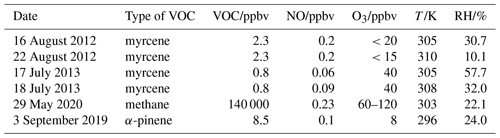In total four experiments investigating the oxidation of myrcene by OH were performed (Table 1), two of which were done at medium levels of nitric oxide (NO) ranging from 0.1 to 0.4ppbv (on 18 August 2012, , from 0.18 to 0.43 ppbv of NO, Fig. 5, and on 22 August 2012, , from 0.15 to 0.30 ppbv of NO, Fig. S1 in the Supplement), while lower NO mixing ratios below 0.11ppbv were achieved in the other two experiments (17 July 2013, , Fig. S2 in the Supplement, and 18 July 2013, , Fig. S3 in the Supplement).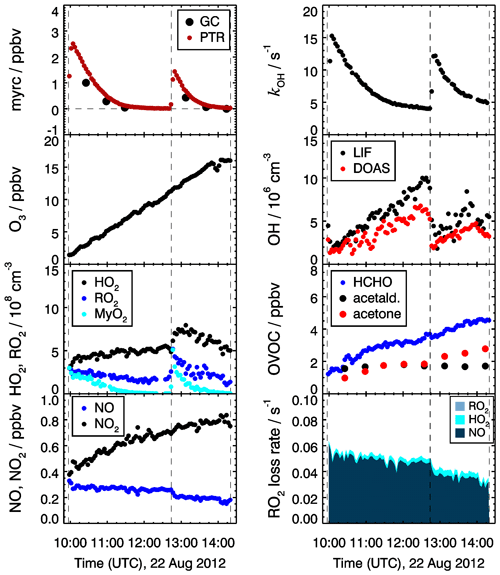Figure 5Trace gas and radical concentrations in the chamber experiment investigating the OH oxidation of myrcene (myrc) at NO mixing ratios between 200 and 300 pptv on 22 August 2012. Peroxy radicals from the reaction of OH with myrcene (MyO2) and total organic peroxy radical (${\mathrm{RO}}_{\mathrm{2}}={\mathrm{MyO}}_{\mathrm{2}}+{\mathrm{RO}}_{\mathrm{2},\mathrm{other}}$) concentrations are calculated from observed radical concentrations as explained in Sect. 3.4. In the lowest right panel, the loss rates of total RO2 with respect to their reaction with NO and radical recombination reactions are shown.

The experimental procedure was similar in all experiments. The chamber was cleaned in the night before the experiment by flushing the chamber with a high flow of synthetic air to remove all trace gases from previous experiments. Figure 5 shows, as an example, time series of trace gases for the experiment conducted on 22 August 2012. Experiments started in the morning with humidification of the chamber air in the dark. This was achieved by flushing evaporated Milli-Q water into the chamber together with a high flow of synthetic air. The chamber air was exposed to sunlight approximately for 1 h without the presence of any additional reactant to determine the source strengths of chamber sources for formaldehyde for the specific experiment. In the experiments in 2013, approximately 50 ppbv of ozone produced by a silent discharge ozonizer (O3onia) was injected to suppress NO in the reaction with O3. Products from the ozonolysis of myrcene are similar to the products formed from the reaction with OH. These could lead to systematic errors in the conclusions with respect to the OH oxidation scheme. Given the typical OH concentration in this study (5×106cm−3), the contribution of ozonolysis to the entire oxidation of myrcene is less than 20 %. The potential interfering is minimized in the medium NO cases, where ozonolysis only contributes 10 % of the myrcene oxidation.

Air mixtures of myrcene (Sigma-Aldrich, purity 99 %) were premixed in a canister (stainless steel with SilcoNert coating). The mixture was injected into the chamber air by calibrated mass flow controllers to reach myrcene mixing ratios of several parts per billion by volume. Injections were performed two times (approximately 2 ppbv each) in experiments with medium NO, and four injections with smaller concentrations (approximately 1 ppbv) were performed in the other experiments.

Additional reference experiments were performed using the same procedure like for the experiments with myrcene, but either with one injection of 140 ppmv of methane (29 May 2020, , Fig. S4 in the Supplement, and 10 July 2013) or three injections of 8.5 ppbv of α-pinene (3 September 2019, , Fig. S5 in the Supplement). These experiments were used to evaluate the accuracies of the procedures that were used to analyse the organic nitrate formation and radical budgets in the experiments with myrcene.

## 3.2 Measurement of trace gas concentrations

Table 2 summarizes properties of instruments used in this work. The set of instruments was similar to that used in previous experiments investigating the photochemistry of organic compounds . Therefore, only a brief description is given here. Ozone was detected by a UV photometer (Ansyco). NO concentrations were measured by a chemiluminescence instrument (Eco Physics) that also detected nitrogen dioxide (NO2) after conversion to NO in a photolytic converter. Methane, water vapour and carbon monoxide concentrations were measured by a cavity ring-down instrument (Picarro). Nitrous acid (HONO) was detected by a custom-built long-path absorption photometry (LOPAP) . Photolysis frequencies were derived from solar actinic flux measurements by a spectroradiometer outside the chamber. Calculations take into account the reduction of radiation by the chamber construction elements and the Teflon film .

Table 2Instrumentation for radical and trace gas detection in the experiments.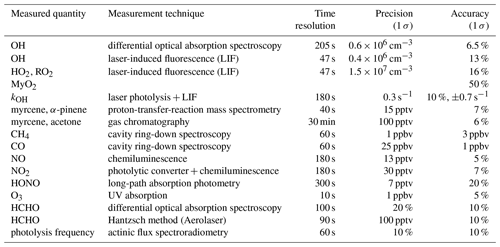Myrcene was detected by gas chromatography coupled with a flame ionization detector (GC-FID) and by a proton-transfer-reaction mass spectrometer (PTR-MS, Ionicon). The PTR-MS was not calibrated for myrcene. Therefore, the signal was scaled to match the GC-FID measurements and used because of its higher time resolution (40 s) compared to that of GC-FID (30 min). Acetone and acetaldehyde were also measured by GC-FID. Formaldehyde (HCHO) measurements were performed by a Hantzsch instrument (Aerolaser) or by differential optical absorption spectrometry (DOAS). The HCHO concentrations measured by the different methods has been shown to agree within 10 % in a series of experiments in the SAPHIR chamber, in which both instruments concurrently measured . OH reactivity (kOH), which is the pseudo-first-order rate constant of the OH radical loss, was measured by a laser flash photolysis–laser-induced fluorescence instrument .

## 3.3 Measurement of radical concentrations

Measurements of OH radicals were performed by differential optical absorption spectrometry (DOAS) and laser-induced fluorescence (LIF) (, and references therein). The DOAS instrument was not available in the experiment on 16 August 2012. In the experiment 6 d later (22 August 2012), OH concentrations measured by the LIF instrument were consistently 20 % higher than those measured by DOAS, which is considered to be an absolute standard. The difference is larger than the combined 1σ uncertainty (14.5 %) of the measurements and is probably caused by a calibration error of the LIF instrument. This assumption is supported by the observed decay of myrcene, which is caused by the reaction with OH. The decay can be accurately described with LIF data if these are reduced by 20 % in both experiments (Fig. S6 in the Supplement, Sect. 6.1). Therefore, OH measurements by LIF were scaled by a factor of 0.8 for both experiments. For the experiments in 2013, OH measurements by LIF and DOAS agreed well within 5 %. For the analysis of this work, OH measurements by the DOAS instrument are used if available. Interference could occur in OH measurements by the LIF instrument from alkene ozonolysis at exceptionally high concentrations of reactants . However, the ozone and myrcene concentrations used in this study were much lower compared to concentrations used in the characterization experiments in . Therefore, it is not expected that similar interferences were significant for measurements in these experiments. Thus, the observed differences in the OH measurements of the LIF and DOAS instruments were most likely caused by calibration errors.

In addition to OH, peroxy radicals (HO2 and RO2) can be detected by the LIF instrument after chemical conversion to OH. The conversion of HO2 radicals is accomplished by adding excess NO in a second low-pressure fluorescence cell, in which the sum of HO2 and OH concentrations is measured . The measurement of RO2 is accomplished in a two-stage system (ROxLIF) in which all atmospheric ROx radicals are first converted to HO2 by added NO and CO in a flow reactor . This is followed by HO2-to-OH conversion with additionally added NO in a fluorescence cell. In this case, RO2 is determined as the difference of ROx and OH+HO2. The operational parameters of the reactor are optimized to maximize the detection sensitivity for methyl peroxy radicals (CH3O2). However, it was found in previous studies that specific RO2 species from other VOCs may be converted less efficiently in the reactor if their conversion to HO2 needs more reaction steps and therefore more reaction time than in the case of CH3O2 . An alternative explanation for the lower sensitivity could be the reversibility of the MyO2 formation at low O2 in the reactor, which slow down the conversion to HO2.

Table 3Chemical reactions considered in the radical budget analysis of OH, HO2 and RO2. Some reactions are only applicable in the experiment with either methane, α-pinene or myrcene, in which specific RO2 radicals, CH3O2, MyO2 or APO2 are formed. RO2+RO2 reactions are not listed, because they do not significantly contribute to the loss of radicals for conditions of experiments here.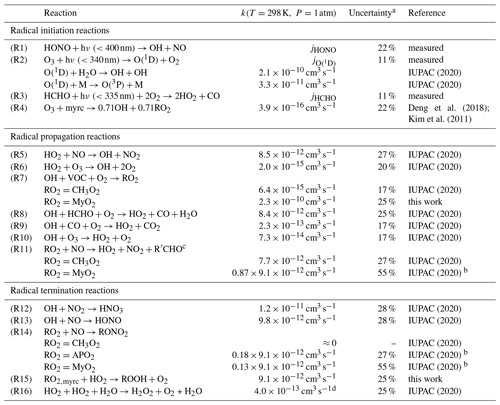a Uncertainty measurements (Table 2) and kinetic rate constants (10 % for reactions of inorganic and methyl peroxy radicals and 15 % for other). b Reaction rate constant: ; the branching ratio of 0.87 is taken from this work. c The dominant reaction of alkoxy radical is to form HO2, which is not rate limiting for RO2-to-HO2 conversion. d Effective reaction rate constant for 1 % H2O mixing ratio.

For the evaluation of data in this work, the ROxLIF instrument was calibrated for CH3O2 and myrcene RO2 radicals (MyO2) that were produced by reaction of OH with the corresponding VOC in the radical calibration source as described by . The detection sensitivity was only half as high for MyO2 compared to CH3O2. The lower sensitivity could hint that some specific MyO2 isomers are not efficiently converted to HO2 for conditions inside the conversion reactor. The difference in the detection sensitivity for MyO2 and other RO2 species like CH3O2 adds to the uncertainty of measurements, because a mixture of different peroxy radicals is present during the experiments and the exact distribution of RO2 species is not known. For the analysis in this work, the fraction of MyO2 is estimated as described in the next subsection, in order to account for the lower sensitivity.

## 3.4 VOC reactivity and RO2 speciation in myrcene experiments

The total OH reactivity (kOH) that was measured in the experiments consists of reactivity from organic and inorganic compounds. Total OH reactivity was measured by LP-LIF, while the speciated OH reactivity for individual species X was calculated from the products of concentrations of X and its reaction rate vs. OH. The reaction of OH with most of the organic compounds leads to the formation of RO2 radicals. Therefore, it is useful to distinguish between OH reactivity from those compounds unmeasured (kOHVOC), and OH reactivity calculated from measured concentrations of inorganic compounds (CO, NO, NO2) and formaldehyde:

$\begin{array}{ll}{k}_{\mathrm{OHVOC}}=& \phantom{\rule{0.25em}{0ex}}{k}_{\text{OH}}-\left({k}_{\mathrm{8}}\left[\text{HCHO}\right]+{k}_{\mathrm{9}}\left[\text{CO}\right]+{k}_{\mathrm{12}}\left[{\mathrm{NO}}_{\mathrm{2}}\right]\\ \text{(1)}& & \phantom{\rule{0.25em}{0ex}}+{k}_{\mathrm{13}}\left[\text{NO}\right]\right),\end{array}$

where ki represents the bimolecular rate constants of reactions Ri listed in Table 3. For the reference experiment with methane, only methane contributed to the VOC reactivity, because its oxidation product is formaldehyde, which does not produce RO2 in the reaction with OH. Therefore, methyl peroxy radicals are the only RO2 radical expected in that experiment. For the experiments with myrcene, the VOC reactivity includes the reactivity from myrcene and from partly unmeasured oxygenated organic compounds (OVOC) that are products of the myrcene oxidation. The OH reactivity from myrcene (kOH myrcene) can be calculated from measured myrcene concentrations and the rate constant of its reaction with OH (k7, Table 3):

$\begin{array}{}\text{(2)}& {k}_{\text{OH myrcene}}={k}_{\mathrm{7}}\left[\text{myrcene}\right].\end{array}$

Assuming that each OH reaction with an organic compound except formaldehyde in the experiments in this work leads to the formation of one RO2 radical and all RO2 radicals have similar chemical lifetimes, the distribution of RO2 species from different VOCs is similar to the distribution of the OH reactivity from the VOCs. Therefore, the concentration of RO2 derived from myrcene (MyO2) can be approximated by scaling the total measured organic peroxy radical concentration ([RO2]m) that is determined assuming the same instrument sensitivity for all RO2 with the ratio of OH reactivity from myrcene (kOH myrcene) to the total OH reactivity from VOCs (kOH VOC). Taking also into account that the sensitivity of the instrument for MyO2 (${S}_{{\mathrm{MyO}}_{\mathrm{2}}}$) is reduced compared to the sensitivity for CH3O2 (SCH3O2), the concentration of MyO2 can be calculated from observed quantities:

$\begin{array}{}\text{(3)}& \left[{\mathrm{MyO}}_{\mathrm{2}}\right]=\frac{\mathrm{1}}{\frac{{S}_{\mathrm{MyO}\mathrm{2}}}{{S}_{\mathrm{CH}\mathrm{3}\mathrm{O}\mathrm{2}}}+\frac{{k}_{\text{OH VOC}}}{{k}_{\text{OH myrcene}}}-\mathrm{1}}\left[{\mathrm{RO}}_{\mathrm{2}}{\right]}_{m}.\end{array}$

RO2 species originating from other hydrocarbons than myrcene (RO2,other) can be calculated using the remaining fraction of the VOC reactivity:

$\begin{array}{}\text{(4)}& \left[{\mathrm{RO}}_{\mathrm{2},\mathrm{other}}\right]=\left[{\mathrm{MyO}}_{\mathrm{2}}\right]\left(\frac{{k}_{\text{OH VOC}}}{{k}_{\text{OH myrcene}}}-\mathrm{1}\right).\end{array}$

Equations (3) and (4) allow calculating a more realistic total RO2 concentration by taking the sum of MyO2 and RO2,other instead of using the measured RO2 concentration if the same instrument sensitivity for all RO2 is assumed. An example of the result of this calculation is shown in Fig. 5, demonstrating that MyO2 was the dominant RO2 species right after each myrcene injection. However, these values have a high uncertainty that cannot easily be quantified because, for example, the detection sensitivity for RO2 produced in the reaction of OH with oxidation products may also differ from that of methyl peroxy radicals. The uncertainty of calculated RO2 concentrations is lowest after each injection of myrcene, because the total OH reactivity and therefore RO2 production are dominated by myrcene. Therefore, the analysis of radical production and destruction rates in this work (Sect. 6.4) focuses on the times right after each myrcene injection.

4 Experimental determination of organic nitrate yields

The determination of organic nitrate yields from photochemical VOC oxidation makes use of a budget analysis of the sum of NOx and HONO in the chamber air. In the following, the sum of NO, NO2 and HONO concentrations is defined as NO${}_{y}^{*}$, which can be calculated from measurements of these species (Table 2).

In the sunlit SAPHIR chamber, the only source of reactive nitrogen is the emission of HONO from the chamber film (Sect. 3.1). The source strength Q(HONO) is variable and depends on solar ultraviolet radiation, temperature and relative humidity . HONO is photolysed to OH and NO (Reaction R1), which is further oxidized to NO2 by reactions with O3, HO2 (Reaction R5) and RO2 (Reaction R11). The sum of NO${}_{y}^{*}$ is chemically lost in the chamber by reactions forming nitric acid (HNO3) (Reaction R12) and organic nitrates (RONO2) (Reaction R14). In addition, the NO${}_{y}^{*}$ species are removed ($L\left({\mathrm{NO}}_{y}^{*}\right)$) by transport due to the replenishment flow that compensates for chamber leakage and gas sampling of analytical instruments with a first-order rate constant of ${k}_{\mathrm{d}}\approx \mathrm{1.6}×{\mathrm{10}}^{-\mathrm{5}}$s−1 (Sect. 3.1):

$\begin{array}{}\text{(5)}& L\left({\mathrm{NO}}_{y}^{*}\right)=\left(\left[\mathrm{NO}\right]+\left[{\mathrm{NO}}_{\mathrm{2}}\right]+\left[\mathrm{HONO}\right]\right){k}_{\mathrm{d}}.\end{array}$

The dilution rate is monitored by the input flow rate of synthetic air, which yields an accuracy of kd better than 1 %. Hence, the uncertainty due to the dilution is neglected due to its small contribution. The concentration of NO${}_{y}^{*}$ at a given time is then determined by the difference between the time-integrated production and loss terms.

$\begin{array}{ll}\left[{\mathrm{NO}}_{y}^{*}\right]=& \phantom{\rule{0.25em}{0ex}}\underset{t}{\int }\left(Q\left(\text{HONO}\right)-{k}_{\mathrm{12}}\left[\mathrm{OH}\right]\left[{\mathrm{NO}}_{\mathrm{2}}\right]-{k}_{\mathrm{14}}\left[{\mathrm{RO}}_{\mathrm{2}}\right]\left[\mathrm{NO}\right]\\ \text{(6)}& & \phantom{\rule{0.25em}{0ex}}-L\left({\mathrm{NO}}_{y}^{*}\right)\right)\mathrm{d}t\end{array}$

It is assumed that the formations of HNO3 and RONO2 are effective sinks for NOx and that reformation of NOx by its reactions with OH or photolysis does not play a role. This assumption is justified because the low reaction rate constant with OH and small absorption cross sections of HNO3 and RONO2 lead to their lifetimes in the range of several days, which is much slower than the timescale of the experiments. The loss of NO2 due to reaction with ozone which forms nitrate radicals (NO3) is neglected in Eq. (6), because the NO3 radical is efficiently converted back to NO2 by photolysis and reaction with NO in the photo-oxidation experiments in this work. The formation of other oxidized nitrogen species such as peroxy nitric acid (HNO4) and acetyl peroxy nitrate (PAN) is also assumed to be negligible because their mixing ratios are expected to be only a few tens of parts per trillion by volume for conditions of the experiments.

The formation rate of HONO that is within the range of a few hundred parts per trillion by volume per hour in the chamber experiments can be determined from measurements of OH, NO, HONO and j(HONO) by assuming a photo-stationary state for the HONO concentration:

$\begin{array}{ll}\frac{\mathrm{d}\left[\text{HONO}\right]}{\mathrm{d}t}=& \phantom{\rule{0.25em}{0ex}}Q\left(\text{HONO}\right)-{j}_{\text{HONO}}\left[\text{HONO}\right]\\ & \phantom{\rule{0.25em}{0ex}}+{k}_{\mathrm{OH}+\mathrm{NO}}\left[\text{OH}\right]\left[\text{NO}\right]=\mathrm{0}\\ \text{(7)}& Q\left(\text{HONO}\right)=& \phantom{\rule{0.25em}{0ex}}{j}_{\text{HONO}}\left[\text{HONO}\right]-{k}_{\mathrm{13}}\left[\text{OH}\right]\left[\text{NO}\right].\end{array}$

For the experimental conditions ($j\left(\text{HONO}\right)=\mathrm{8}×{\mathrm{10}}^{-\mathrm{4}}$s−1) the photo-stationary state is reached within approximately 20 min. On this timescale, the dilution of HONO by the chamber replenishment flow is only 2 % and is therefore neglected. The uncertainty of the HONO formation rate (Eq. 7) is dominated by the uncertainty in the HONO measurement (22 %, Table 3).

Using measured concentrations of NO, NO2 and HONO, the concentration of NO that is converted to organic nitrates during the experiment (ΔRONO2) is determined by the balance of nitrogen oxide concentrations:

$\begin{array}{ll}& \mathrm{\Delta }\left[{\mathrm{RONO}}_{\mathrm{2}}\right]\phantom{\rule{0.25em}{0ex}}=\underset{t}{\int }{k}_{\mathrm{14}}\left[{\mathrm{RO}}_{\mathrm{2}}\right]\left[\mathrm{NO}\right]\mathrm{d}t\\ & \phantom{\rule{1em}{0ex}}={\mathrm{\Phi }}_{\mathrm{RONO}\mathrm{2}}\underset{t}{\int }\left({k}_{\mathrm{11}}+{k}_{\mathrm{14}}\right)\left[{\mathrm{RO}}_{\mathrm{2}}\right]\left[\mathrm{NO}\right]\mathrm{d}t\\ & \phantom{\rule{1em}{0ex}}=\underset{t}{\int }\left(Q\left(\text{HONO}\right)-{k}_{\mathrm{12}}\left[\text{OH}\right]\left[{\mathrm{NO}}_{\mathrm{2}}\right]\\ \text{(8)}& & \phantom{\rule{1em}{0ex}}-L\left({\mathrm{NO}}_{y}^{*}\right]\right)\right)\mathrm{d}t-\left(\left[\text{NO}\right]+\left[{\mathrm{NO}}_{\mathrm{2}}\right]+\left[\text{HONO}\right]\right).\end{array}$

The reaction yield of organic nitrates (ΦRONO2) can be then derived as slope of a linear fit (Eq. 8). The accuracy of the yield is mainly determined by the accuracy the kinetic reaction rate constants and measurements that vary between the specific experiments.

## 4.1 Test experiments with methane and α-pinene

In order to test the method described above, organic nitrate yields from the photo-oxidation of methane and α-pinene were experimentally determined and compared with literature values. NO mixing ratios were between 100 and 300 pptv in both experiments, so that at least 60 % of the RO2 radicals reacted with NO.

Figure 6 shows time series of NOy species in α-pinene and methane experiments. In the methane experiment, the total mixing ratio of NOy species calculated from the HONO chamber source (Eq. 7) is around 1.3 ppbv and is mainly explained by measured NO2 and NO concentrations. In the experiment with α-pinene, the calculated NOy mixing ratio increased from 1 to 1.3 ppbv within 2 h. Approximately 1 ppbv was present as NO2 and NO and the remaining fraction can be attributed to the formation of organic nitrates from α-pinene. The alkyl nitrate yield is derived from Eq. (8) by a linear fit as described above.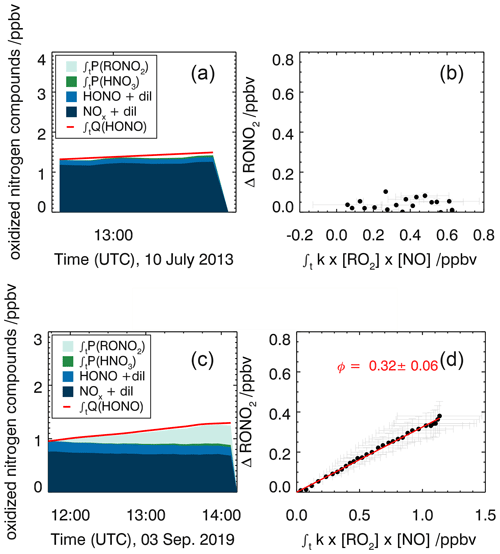Figure 6Time series of nitrogen oxide species in the experiments with methane (10 July 2013, a) and α-pinene (3 September 2019, c). The red lines in panels (a) and (c) are time-integrated HONO emissions calculated as described in the text. NOx and HONO concentrations were measured; the time-integrated NO2 loss by HNO3 formation from the reaction of NO2 with OH and the time-integrated NO2 loss by RONO2 formation from the reaction of RO2 with NO were calculated as described in the text. The alkyl nitrate yield (ΦRONO2) is determined from the regression analysis (red line, d) using Eq. (8). Errors are derived from error propagation of measurements.

For the experiment with methane, the alkyl nitrate yield is smaller than the error of the calculation (0.00±0.04, Fig. 6). This small value agrees with literature values of <0.003 and 0.0039±0.0011 at room temperature.

The alkyl nitrate yield determined from the OH oxidation experiment with α-pinene is 0.32±0.06. The accuracy is mainly due to the uncertainty of RO2 measurements. Reported literature values are 0.01 , 0.18±0.09 and 0.26±0.07 . All experiments were conducted at measurement conditions where the reaction of RO2 with NO was the dominant RO2 reaction pathway, so that the obtained organic nitrate yields can be attributed to the yield from reaction of α-pinene-derived RO2. The value determined in this work agrees well with values determined from direct measurements using FT-IR spectroscopy in experiments by and within the specified errors. In contrast, approximated the nitrate yield from mass spectrometer measurements with a high uncertainty that likely explains the much lower yield in their experiments compared to the other two studies and results in this work.

The good agreement between nitrate yields determined in the experiments with methane and α-pinene with reported values in literature demonstrates that the applied method for the determination of alkyl nitrate formation in the chamber gives reliable results.

5 Experimental analysis of the chemical budgets of OH, HO2, RO2 and ROx

## 5.1 Radical production, destruction and regeneration reactions

In the chamber experiments, OH, HO2 and RO2 radicals are primarily formed from photolysis of HONO (Reaction R1), O3 (Reaction R2), formaldehyde (Reaction R3) and from the ozonolysis of myrcene (Reaction R4). Radical termination processes include the formation of nitrates (HNO3, HONO and RONO2, Reactions R12, R13 and 14) and peroxides (ROOH and H2O2, Reactions R15 and R16). The contribution from RO2 self-combination reactions is negligible and not considered here. The photolysis of peroxides that can lead to the production of radicals is neglected due to the slow photolysis frequency (typical value for example for CH3OOH: $\mathrm{2}×{\mathrm{10}}^{-\mathrm{6}}$s−1 at noontime). The rates for the primary production of ROx radicals (P(ROx)) and for the termination loss rate (L(ROx)) can be calculated as

$\begin{array}{ll}& P\left({\mathrm{RO}}_{x}\right)=\phantom{\rule{0.25em}{0ex}}{j}_{\text{HONO}}\left[\text{HONO}\right]+{\mathrm{\Phi }}_{\mathrm{OH},\mathrm{2}}{j}_{\mathrm{O}{\left(}^{\mathrm{1}}\mathrm{D}\right)}\left[{\mathrm{O}}_{\mathrm{3}}\right]\\ & \phantom{\rule{1em}{0ex}}+\mathrm{2}{j}_{\mathrm{HCHO}}\left[\mathrm{HCHO}\right]+\left({\mathrm{\Phi }}_{\mathrm{OH},\mathrm{4}}+{\mathrm{\Phi }}_{{\mathrm{RO}}_{\mathrm{2}},\mathrm{4}}\right)\\ \text{(9)}& & \phantom{\rule{1em}{0ex}}×{k}_{\mathrm{4}}\left[\text{VOC}\right]\left[{\mathrm{O}}_{\mathrm{3}}\right],& L\left({\mathrm{RO}}_{x}\right)=\phantom{\rule{0.25em}{0ex}}\left({k}_{\mathrm{12}}\left[{\mathrm{NO}}_{\mathrm{2}}\right]+{k}_{\mathrm{13}}\left[\text{NO}\right]\right)\left[\text{OH}\right]\\ \text{(10)}& & \phantom{\rule{1em}{0ex}}+\left({k}_{\mathrm{14}}\left[\text{NO}\right]+\mathrm{2}{k}_{\mathrm{15}}\left[{\mathrm{HO}}_{\mathrm{2}}\right]\right)\left[{\mathrm{RO}}_{\mathrm{2}}\right]+\mathrm{2}{k}_{\mathrm{16}}\left[{\mathrm{HO}}_{\mathrm{2}}{\right]}^{\mathrm{2}}.\end{array}$

For the calculation of P(ROx) and L(ROx), measured radical and trace gas concentrations are used. For the analysis of the myrcene experiments, total RO2 concentrations include corrections for the reduced detection sensitivity of MyO2 as explained in Sect. 3.4. The applied rate constants are listed in Table 3. The OH and RO2 yields are taken from . In their work, the yield of HO2 is zero. The lumped rate constant for the reactions between RO2 and HO2 (Reaction R15) has a rather large uncertainty, because rate constants of different RO2 species can be different by up to a factor of 4 . The uncertainty of other reaction rate constants is typically around 10 %. In addition, the accuracies of measurements (Table 2) add to the total uncertainty in the calculation of loss and production rates. In these experiments, there is a small background OH reactivity (<1s−1) which can be a permanent loss of radicals but could also regenerate HO2 and/or RO2. However, this background reactivity is small compared to the OH reactivity from methane and myrcene during the experiments (>15s−1), so that it does not affect the analysis.

If production and destruction rates of single ROx species (OH, HO2 and RO2) are calculated, radical conversion reactions need to be additionally taken into account. The total OH loss rate (L(OH)) can be quantified by the product of measured OH concentrations and total OH reactivity:

$\begin{array}{}\text{(11)}& L\left(\text{OH}\right)=\left[\text{OH}\right]{k}_{\text{OH}}.\end{array}$

The OH production rate (P(OH)) can be calculated from the sum of production from HONO (Reaction R1) and O3 photolysis followed by water reaction (Reaction R2), ozonolysis of VOCs (Reaction R4) and radical regeneration from HO2 reacting with NO (Reaction R5) or O3 (Reaction R6):

$\begin{array}{ll}P\left(\text{OH}\right)=& \phantom{\rule{0.25em}{0ex}}{j}_{\text{HONO}}\left[\text{HONO}\right]+{\mathrm{\Phi }}_{\mathrm{OH},\mathrm{2}}{j}_{\mathrm{O}{\left(}^{\mathrm{1}}\mathrm{D}\right)}\left[{\mathrm{O}}_{\mathrm{3}}\right]\\ & \phantom{\rule{0.25em}{0ex}}+{\mathrm{\Phi }}_{\mathrm{OH},\mathrm{4}}{k}_{\mathrm{4}}\left[\text{VOC}\right]\left[{\mathrm{O}}_{\mathrm{3}}\right]\\ \text{(12)}& & \phantom{\rule{0.25em}{0ex}}+\left({k}_{\mathrm{5}}\left[\text{NO}\right]+{k}_{\mathrm{6}}\left[{\mathrm{O}}_{\mathrm{3}}\right]\right)\left[{\mathrm{HO}}_{\mathrm{2}}\right].\end{array}$

The loss of HO2 (L(HO2)) and RO2 (L(RO2)) radicals are dominated by their reactions with NO for conditions of the experiments here (Reactions R5, R11, R14) in addition to recombination of peroxy radicals including reactions of HO2 with RO2 (Reaction R15) and HO2 self-reactions (Reaction R16):

$\begin{array}{ll}L\left({\mathrm{HO}}_{\mathrm{2}}\right)=& \phantom{\rule{0.25em}{0ex}}\left({k}_{\mathrm{5}}\left[\text{NO}\right]+{k}_{\mathrm{6}}\left[{\mathrm{O}}_{\mathrm{3}}\right]+{k}_{\mathrm{15}}\left[{\mathrm{RO}}_{\mathrm{2}}\right]\\ \text{(13)}& & \phantom{\rule{0.25em}{0ex}}+\mathrm{2}{k}_{\mathrm{16}}\left[{\mathrm{HO}}_{\mathrm{2}}\right]\right)\left[{\mathrm{HO}}_{\mathrm{2}}\right],\text{(14)}& L\left({\mathrm{RO}}_{\mathrm{2}}\right)=& \phantom{\rule{0.25em}{0ex}}\left(\left({k}_{\mathrm{11}}+{k}_{\mathrm{14}}\right)\left[\text{NO}\right]+{k}_{\mathrm{15}}\left[{\mathrm{HO}}_{\mathrm{2}}\right]\right)\left[{\mathrm{RO}}_{\mathrm{2}}\right].\end{array}$

The HO2 production (P(HO2)) rate can be calculated from the photolysis of aldehydes, of which HCHO (Reaction R3) were measured in these experiments, and reactions that convert OH or RO2 to HO2. OH-to-HO2 conversion occurs in the reaction of OH with HCHO (Reaction R6), CO (Reaction R7) and O3 (Reaction R8). The reaction of RO2 with NO (Reaction R9) produces either HO2 or organic nitrates (Reaction R14). The total HO2 production rate is then calculated as

$\begin{array}{ll}P\left({\mathrm{HO}}_{\mathrm{2}}\right)=& \phantom{\rule{0.25em}{0ex}}\mathrm{2}{j}_{\text{HCHO}}\left[\text{HCHO}\right]+\left({k}_{\mathrm{6}}\left[\text{HCHO}\right]+{k}_{\mathrm{7}}\left[\text{CO}\right]\\ \text{(15)}& & \phantom{\rule{0.25em}{0ex}}+{k}_{\mathrm{8}}\left[{\mathrm{O}}_{\mathrm{3}}\right]\right)\left[\text{OH}\right]+{k}_{\mathrm{11}}\left[\text{NO}\right]\left[{\mathrm{RO}}_{\mathrm{2}}\right].\end{array}$

RO2 primary production consists of the ozonolysis of VOCs (Reaction R4). In addition, RO2 is produced from radical propagation reactions via the reaction of OH with VOCs. The total production rate (P(RO2)) can be calculated from the VOC reactivity, kOHVOC (Eq. 1), assuming that each reaction of a VOC with OH produces one RO2 radical:

$\begin{array}{}\text{(16)}& P\left({\mathrm{RO}}_{\mathrm{2}}\right)={\mathrm{\Phi }}_{\mathrm{RO}\mathrm{2}}{k}_{\mathrm{4}}\left[\text{VOC}\right]\left[{\mathrm{O}}_{\mathrm{3}}\right]+{k}_{\text{OHVOC}}\left[\text{OH}\right].\end{array}$

## 5.2 Radical production and destruction in a test experiment with methane

The chemical radical budget analysis in SAPHIR experiments was tested in a photo-oxidation experiment with methane. The chemical oxidation mechanism of methane is much simpler than that of monoterpenes. (1) Ozonolysis reactions do not play a role. (2) Organic peroxy radicals that are formed in the chemical mechanism are methyl peroxy radicals (CH3O2), which can be accurately measured by the ROxLIF system. (3) There are recommendations for rate constants for reactions involving methyl peroxy radicals (IUPAC2020).

Reaction rates for the methane oxidation experiment (29 May 2020) are shown in Fig. 7. Since no DOAS OH measurements were available for this experiment, OH measurements by the LIF instrument were used (Fig. S4 in the Supplement). Total turnover rates are similar for all single radical species (OH, HO2, RO2) with values between 8 and 12 ppbv h−1 and do not vary much over the course of the experiment. The loss of peroxy radicals is dominated by the radical regeneration reaction with NO, whereas radical recombination reactions contribute less than 10 % to the entire loss of peroxy radicals. OH is nearly only lost by its reaction with methane and formaldehyde.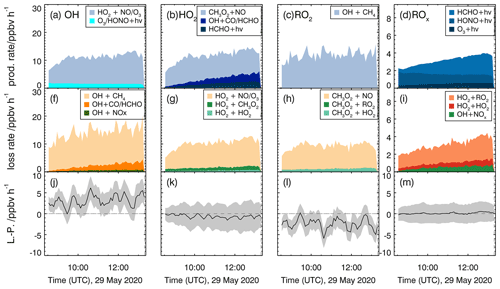Figure 7Calculated production and destruction rates of reactions involving radicals for the reference experiment with methane (29 May 2020). Production rates for OH, HO2, RO2 and total ROx are shown in panels (a)(d), respectively, and loss rates for OH, HO2, RO2 and total ROx are shown in panels (f)(i). Lower panels (j–m) show the differences between loss and production rates (LP) with the associated accuracy (grey areas).

The production and destruction rates of total ROx are significantly smaller than those of single radical species with values that rise from 2 to 4 ppbv h−1 over the course of the experiment as radical regeneration reactions cancel out. The increase is due to radicals from the photolysis of formaldehyde that are continuously produced from the chamber wall (Sect. 3.1) and in the reaction of OH+ methane. The increase in radical production is balanced by increasing rates of peroxy radical recombination reactions and of the reaction of OH with NO2. The latter is due to the increase in nitrogen oxide concentrations from the chamber source of nitrous acid.

Radical production and destruction rates of each radical species and of total ROx are roughly balanced within the uncertainty of the calculation (Fig. 7). Maximum deviations are less than 0.5 ppbv h−1 for the production and destruction rates of ROx and HO2. Differences are much smaller than the accuracy of the calculation (1.5 ppbv h−1 for ROx and 2 ppbv h−1 for HO2). Higher deviations with values of up to 4 ppbv h−1 are seen for OH and RO2 but opposite behaviour. The accuracy of OH and RO2 radical production and destruction rates is in the range of 2 to 3 ppbv h−1, which cannot explain the discrepancies. As the ROx and HO2 budgets are closed using measured OH concentrations, the imbalance between OH and RO2 production and destruction indicates an unknown systematic error in the conversion rate from OH to RO2. One possible explanation for the observed imbalances would be that the calculated reaction rate of OH+CH4 is too large. This could either be caused (i) by the measured OH or CH4 concentrations being too high or (ii) by the applied reaction rate constant ${k}_{\mathrm{OH}+{\mathrm{CH}}_{\mathrm{4}}}$ being too large.

The methane concentrations in the chamber were above the measurement range specified by the manufacturer (Picarro, 0–20 ppmv). However, an instrumental test of the instrument done prior to the chamber experiment showed that the stated measurement accuracy holds at the concentration (150 ppmv) used in the presented experiment. A small background reactivity of 2 s−1 was found before the injection of methane. The OH reactivity calculated from measured methane concentration and the reaction rate constant is about 26 s−1, which is consistent with the direct OH reactivity measurement considering the contribution of background reactivity (Fig. S4 in the Supplement). This gives the confidence on the accuracy of the methane measurement.

Another reason for the imbalances could be LIF calibration errors that are larger than given in Table 2. The specification for OH in Table 2 was generally confirmed in previous intercomparisons with the OH-DOAS instrument. However, in some instances the LIF measurements were found to deviate more than expected. An example are the experiments on 16 and 22 August 2012 (Figs. 5 and S1 in the Supplement), where the LIF measurements are higher than the DOAS data by a factor 1.2 probably due to an incorrect calibration.

In the following section, radical budgets for the chemical degradation of myrcene are investigated. In these cases, also OH-DOAS data were available, which have a generally better accuracy than LIF. Therefore, the DOAS data were used for the budget analysis (Figs. 1012). Note that LIF and DOAS showed good agreement in these experiments (Figs. S2 and S3 in the Supplement).

The rate constant for OH+CH4 has an uncertainty factor of f=1.1 according to NASA/JPL and f=1.15 according to IUPAC . Thus, the 10 %–15 % difference in the radical budgets would be explainable by a rate constant that is too large.

This partly unresolved discrepancy shows the limitation of the analysis of radical production and destruction rates and needs to be further investigated. The analysis of the experiment with the well-defined chemistry of methane indicates that the maximum accuracy of calculated differences between production and destruction rates for single radical species is 20 % in the chamber experiments.

6 Results and discussion of the experiments with myrcene

## 6.1 Reaction rate constant of the OH reaction with myrcene

The rate constant of the reaction of myrcene with OH is determined from the measured temporal decay of myrcene and the measured OH concentration. The time series of measured myrcene concentrations are compared to calculations using a chemical box model that only includes chemical loss reactions with OH and O3 and dilution. The model is constrained to measured values of temperature, pressure, the dilution rate constant, and OH and O3 concentrations. The initial myrcene concentration is set to the injected amount of myrcene. The reaction rate constant for myrcene with O3 is taken from the work by , who measured a value of $\mathrm{2.21}×{\mathrm{10}}^{-\mathrm{15}}\mathrm{exp}\left(-\left(\mathrm{520}±\mathrm{109}\right)\phantom{\rule{0.125em}{0ex}}\mathrm{K}/T\right)$cm3 s−1 using the relative rate technique. suggested a nearly 20 % faster reaction rate constant of $\mathrm{4.7}×{\mathrm{10}}^{-\mathrm{16}}$cm3 s−1 (T=298K) that is still consistent with the value by within the experimental uncertainties. The chemical lifetime of myrcene with respect to the ozonolysis reaction was approximately 44 min in the low-NOx experiments with O3 concentrations of 40 ppbv but was up to 3 h in the experiments with medium NOx and O3 concentrations of less than 10 ppbv. In comparison, the chemical lifetime of myrcene with respect to the reaction with OH was within the range of 15 min at OH concentrations of approximately 5×106cm−3 observed in all experiments. Hence, the contribution of ozonolysis to the total chemical loss of myrcene in the experiments was 10 % to 26 %.

The rate constant of the reaction of myrcene with OH is optimized, such that the difference between measured and modelled myrcene concentration time series is minimized. For this optimization the OH concentration observed from DOAS is used. This procedure is applied to all three experiments, resulting in a rate constant of $\left(\mathrm{2.3}±\mathrm{0.3}\right)×{\mathrm{10}}^{-\mathrm{10}}$cm3s−1 (T=298K, Table 4, Fig. S6 in the Supplement). The error results mainly from the variability of values determined in the different experiments.

The rate constant determined in this study is in good agreement with those reported in the literature (Table 4). The relative rate technique was used in experiments by and . The value reported by ($\mathrm{3.4}×{\mathrm{10}}^{-\mathrm{10}}$cm3 s−1) is higher than those of this work and reported by but has a high uncertainty of ±35% that is explained by experimental difficulties in the handling of myrcene. The reaction rate constants calculated from structure–activity relationship (SAR) by and give similar results between 1.8 and $\mathrm{1.9}×{\mathrm{10}}^{-\mathrm{10}}$cm3 s−1. Values are approximately 20 % lower than the experimentally derived reaction rate constants. However, differences are within the accuracy of SAR predictions.

## 6.2 Product yields of the reactions of myrcene hydroxy peroxy radicals with NO

When OH reacts with myrcene, about half (48 %) of the OH adds to the $-\mathrm{CH}=\mathrm{C}\left({\mathrm{CH}}_{\mathrm{3}}{\right)}_{\mathrm{2}}$ moiety and the other half (48 %) to the isoprenyl part . In comparison, the SAR developed by predicts a branching ratio of 63:37 for OH addition to the $-\mathrm{CH}=\mathrm{C}\left({\mathrm{CH}}_{\mathrm{3}}{\right)}_{\mathrm{2}}$ and the isoprenyl part, consistent with the values reported in . In this work, the branching ratio is adapted from to be consistent with other studies. In the first case, 4-vinyl-4-pentenal and acetone are formed from the reaction of MyO2 with NO (Sect. 2). In the second case, 2-methylidene-6-methyl-5-heptenal or 1-vinyl-5-methyl-4-hexenone is produced together with formaldehyde. Thus, the yields of acetone and formaldehyde are indicators for the yields of the OH addition to myrcene.

Acetone and formaldehyde yields are calculated from measured time series of product species and myrcene in the experiments on 16 and 22 August 2012, when NO mixing ratios were 200 pptv, so that >90% of RO2 reacted with NO (Fig. 5). Only measurements after the first myrcene injection are used here to avoid that secondary chemistry impacts the yield determination. The two other experiments are not considered for three reasons. The large amount of ozone (up to 50 ppbv) would require considerable correction for myrcene ozonolysis. Second, due to the lower NO concentration, reactions of RO2 with HO2 would be competitive with the reaction of RO2 with NO. Third, the smaller amount of injected myrcene produced fewer oxidation products.

Corrections are applied to measured myrcene, formaldehyde and acetone time series, in order to relate myrcene that reacted with OH and product species that are chemically formed from this reaction following the procedure described in previous work . Product concentrations are corrected for losses due to dilution, photolysis and reaction with OH. In addition, formation of formaldehyde and acetone from chamber sources needs to be subtracted from the measured concentrations. Myrcene concentrations are also corrected for dilution and the fraction of myrcene that reacted with ozone. After corrections have been applied, the relationships between consumed myrcene and product concentrations are linear if species are mainly formed as first-generation products in the reaction of myrcene and OH. The slopes give acetone and formaldehyde yields of 0.45±0.08 and 0.35±0.08, respectively (Fig. 8). The error is caused by the uncertainty in the source strengths of chamber sources for acetone and formaldehyde and the accuracy of measurements.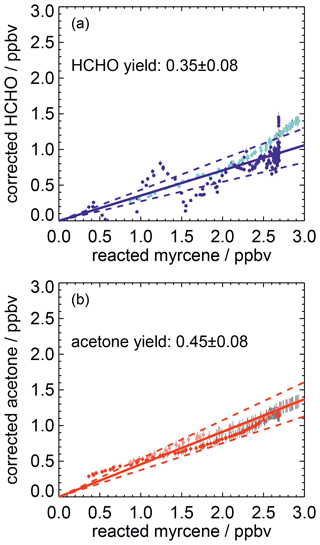Figure 8Corrected product concentrations vs. the myrcene that reacted away with OH for two experiments with medium NO (light colours: 16 August 2012, dark colours: 22 August 2012). Corrections are applied to account for formation of product species not connected to the oxidation of myrcene (chamber sources) and loss processes (reaction with OH, photolysis). The slopes of linear fits (solid lines) give the product yields of HCHO and acetone from the myrcene reaction with OH. The uncertainties of the yield calculations are indicated by dashed lines.

The acetone yield is in excellent agreement with SAR predictions that 48 % of the OH adds to the double bond of the $-\mathrm{CH}=\mathrm{C}\left({\mathrm{CH}}_{\mathrm{3}}{\right)}_{\mathrm{2}}$ moiety . The formaldehyde yield of 0.35±0.08 is lower than the SAR prediction for the OH attack to the isoprenyl part (0.48). One possible reason for the smaller-than-expected formaldehyde yield could be isomerization reactions of the myrcene peroxy radicals shown (Fig. 3), which may not lead to the production of HCHO. The estimated bulk isomerization rates of 0.097 and 0.21 s−1 (Sect. 2) are sufficiently fast to compete with the loss rate of 0.04 s−1 for MyO2 with 200 pptv of NO, so that the reduced formaldehyde yield could be fully explained by isomerization reaction pathways. A different explanation for the lower formaldehyde yield compared to acetone is that the nitrate yield is larger for the MyO2 that would otherwise end up as formaldehyde. In fact, the total product yields is closed to unity (acetone $\left(\mathrm{0.45}±\mathrm{0.08}\right)+\text{formaldehyde}\left(\mathrm{0.35}±\mathrm{0.08}\right)+\text{nitrate}\left(\mathrm{0.13}±\mathrm{0.03}\right)=\mathrm{0.93}±\mathrm{0.12}$).

Acetone and formaldehyde yields agree with published results by and (Table 5). reported a smaller acetone yield of 0.22±0.02 and a significantly higher HCHO yield of 0.74±0.08. However, their HCHO yield carries a large uncertainty because concentrations were outside the range for which the instrument was calibrated.

Table 5Products yields from the reaction of myrcene with OH. NA – not available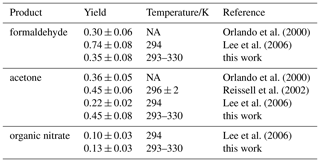The oxygenated organic compound 4-vinyl-4-pentenal has been detected in previous studies from the reaction of myrcene with OH . Fragmentation in the PTR-MS and further oxidation of 4-vinyl-4-pentenal complicated the unambiguous yield determination. Therefore, reported a high yield of 0.4 but also stated a lower limit of 0.09. Acetone is the co-product of 4-vinyl-4-pentenal (Fig. 3). The high limit yield of 0.4 for 4-vinyl-4-pentenal by is therefore consistent with the acetone yield determined in this work. The yield of 4-vinyl-4-pentenal was also measured by , but a lower yield of 0.19±0.04 was found. This low yield is apparently inconsistent with the yield of 0.45±0.06 for the co-product acetone determined in the same experiments (Table 5). The authors suggest that there could be rearrangement of the hydroxyalkoxy radical that competes with the decomposition to 4-vinyl-4-pentenal but may still lead to acetone production, so that the yield for acetone could become higher than that of 4-vinyl-4-pentenal.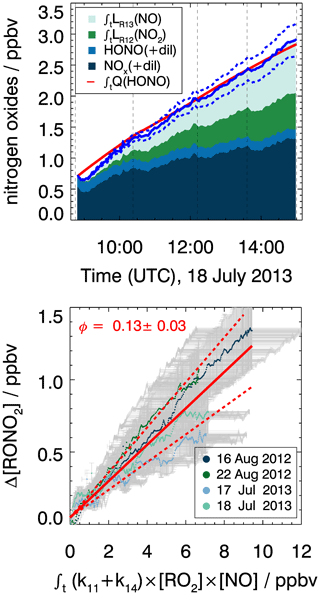Figure 9Determination of the organic nitrate yield from the production of nitrogen oxide species (Q(HONO)) in the chamber experiment. Upper panel: example of cumulated reactive nitrogen oxide mixing ratios over the course of the experiment on 18 July 2013. The blue line denotes the total production of nitrogen oxides including organic nitrate applying a nitrate yield (ΦRONO2) of 0.13. Dashed lines show the error of the total nitrogen oxide calculation. Lower panel: scatter plot of the integrated turnover rate of the reaction of RO2 with NO vs. the unaccounted nitrogen oxide mixing ratios (ΔNOy, Eq. 8). Results from all four experiments are included. The organic nitrate yield is determined by the slope of regression analysis (red line, R2=0.92). Error bars denote experimental uncertainty derived from the accuracy of the measurements (Table 2) and dashed red lines the resulting uncertainty in the slope of the regression analysis.

The yield of organic nitrate from reactions of MyO2 with NO is determined from the analysis of reactive nitrogen oxides in the chamber as described in Sect. 4. This results in an organic nitrate yield of 0.13±0.03 (Fig. 9). This value is consistent with the yield of 0.10±0.03 reported by , who directly measured organic nitrates by mass spectrometry. Values are lower than the yield expected from SAR described in , which predicts a yield of 0.19 that would also apply for other monoterpenes, but may still be within the uncertainty of the SAR predictions. Like for the formaldehyde yield, the smaller-than-predicted organic nitrate yield may also be partly due to competing MyO2 isomerization reactions.

## 6.3 Primary radical production and termination

The primary radical production is due to photolysis (Reactions R1 to R3) and ozonolysis reactions (Reaction R4). Production rates by photolysis of O3, HONO and HCHO are calculated using measured trace gas concentrations and photolysis frequencies. The calculation of the production rate from myrcene ozonolysis requires the knowledge of both the reaction rate constant (Sect. 6.1) and the yield of OH and RO2 radicals. The uncertainty of yields is high. Values range from 0.71 determined from high-level quantum chemistry calculations and kinetic calculations to experimental values of 1.15 with an uncertainty of a factor of 1.5 . It is worth noting that additional radicals could be produced from ozonolysis reactions of oxidation products at later times of the experiment because first-generation organic products from the reaction of myrcene with OH still contain C−C double bonds that can react with ozone.

The radical termination reactions include reactions with nitrogen oxides (Reactions R12–R14) and radical self-reactions (Reactions R15, R16). Loss of radicals in the reaction with NOx and the HO2 self-reaction contributes less than 1 ppbv h−1 to the total loss rate each. Contributions from RO2 self-reactions are expected to be negligible (<2%) because their reaction rate constants are typically much smaller than those of RO2+HO2 reactions (Table 3). The reaction rate constant of the MyO2+HO2 reaction is estimated from SAR by , and, therefore, the value could also have a high uncertainty.

In the experiment with medium NO concentrations (22 August 2012), the total radical production is low, with values of less than 1.5 ppbv h−1, and radical production and destruction are balanced (Fig. 10). No ozone is added, so that radical production from ozonolysis plays a minor role, and radical loss by radical recombination is suppressed due to the competition of peroxy radical reactions with NO. Therefore, the uncertainties in the radical yield of myrcene ozonolysis and the reaction rate constant of the MyO2+HO2 reaction do not impact the results.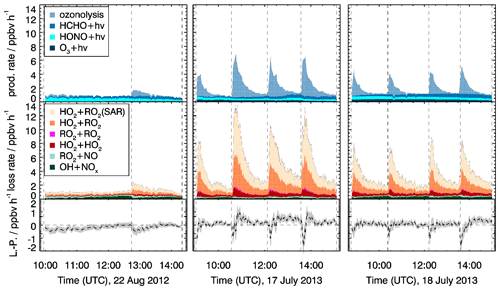Figure 10ROx primary production P (upper panels), termination L (middle panels) rates and their difference (lower panels). The rate constant of the reaction of MyO2 with HO2 was adjusted to minimize the difference between radical production and destruction (see text for more explanation). The HO2+RO2 (SAR) shows the additional radical loss if the unadjusted reaction rate constant is applied. In the bottom row, the lines show the difference between radical destruction and production (LP), with the adjusted rate constant of the reaction of MyO2 with HO2. Grey areas in the lower panels give the uncertainty of LP.

In contrast, radical production and destruction rates in experiments performed at low NO concentrations (17 July 2013, 18 July 2013), which is achieved by suppressing NO in the reaction with O3, are dominated by radical production from myrcene ozonolysis and radical destruction by MyO2+HO2 reactions right after the injection of myrcene. Taking the upper limit of the radical yield from the ozonolysis reaction of 1.42 (0.71 for OH and 0.71 for MyO2), the production is 4 ppbv h−1. The radical primary production could be smaller if the unspecified radical yield of 1.15 suggested by is applied. Applying the reaction rate constant of the MyO2+HO2 derived by SAR (Sect. 2) results in a maximum radical loss rate of 13.5 ppbv h−1 also right after the injection of myrcene when MyO2 concentrations are highest. Because this high destruction rate cannot be balanced by radical production, either the reaction rate constant must be lower than SAR predictions or there are reaction pathways that do not lead to radical termination products (organic peroxide) but regenerate radicals. Due to the high uncertainty of radical production from ozonolysis in these experiments, no firm conclusion can be drawn. The reaction rate constant would need to be reduced between a factor of 0.4 and 0.7 (0.9 to $\mathrm{1.6}×{\mathrm{10}}^{-\mathrm{11}}$cm3 s−1 (T=298K, Table 3) or the yield of radical products would need to be in the range of 0.3 and 0.6 to match the range of radical production.

A reduced MyO2+HO2 reaction rate constant is significantly different from the SAR predictions by . The SAR value is similar for all monoterpenes and agrees well with direct measurements for the reaction rate constant of HO2 with RO2 derived from α-pinene ($\mathrm{2.1}×{\mathrm{10}}^{-\mathrm{11}}$cm3 s−1), γ-terpinene ($\mathrm{2.0}×{\mathrm{10}}^{-\mathrm{11}}$cm3 s−1) and limonene ($\mathrm{2.1}×{\mathrm{10}}^{-\mathrm{11}}$cm3 s−1) reported by . A high yield of radical products from the MyO2+HO2 reaction may not be expected from analogies of isoprene and 2-methyl-2-butene . For these reasons there is no clear conclusion on how exactly rate constants and yields need to be adjusted to balance the ROx production and destruction rates.

## 6.4ROx radical chain propagation reactions

The total production and loss rates of OH, HO2 and RO2 radicals do not exhibit much variability over the course of the experiments with medium NO concentrations (Figs. 11 and S7 in the Supplement). In the experiments with low NO, the turnover rates of radical production and destruction reactions for OH, HO2, and RO2 exhibit peak values when myrcene is injected (Figs. 12 and S8 in the Supplement). This distinct feature is related to the elevated radical production of up to 2 ppbv h−1 from the ozonolysis of myrcene and peak concentrations of peroxy radical concentrations.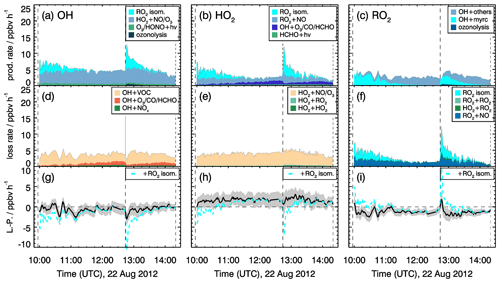Figure 11Rates of radical conversion reactions and imbalances between production and destruction rates (LP) for the experiment with medium NO mixing ratios (0.15 to 0.30 ppbv) on 22 August 2012. Grey areas in the lower panels give the uncertainty of the calculation (LP). In the bottom row, the black and cyan lines denote the radical budget with and without considering MyO2 isomerization reactions. Upper limits for yields of OH and HO2 radicals from MyO2 isomerization reactions of one are assumed in the calculations of their production rates.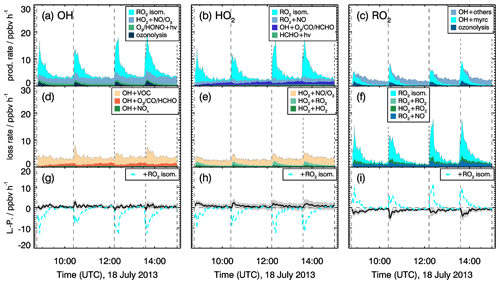Figure 12Rates of radical production and destruction reactions for OH, HO2 and RO2 for experiments at low NO mixing ratio (<0.11ppbv) on 18 July 2013. Grey areas in the lower panels give the uncertainty of LP. In the bottom row, the black and cyan lines denote the radical budget with and without considering MyO2 isomerization reactions. Upper limits for yields of OH and HO2 radicals from MyO2 isomerization reactions of one are assumed in the calculations of their production rates.

Production and destruction rates of OH radicals are balanced within the accuracies of the calculation in all experiments. Rates are similar in all experiments with values around 4 ppbv h−1. Maximum RO2 turnover rates are lower (2 to 3 ppbv h−1) compared to those of OH and HO2, because up to 25 % of the OH radicals are directly converted to HO2, for example in the reaction of OH with CO or HCHO. Because myrcene is consumed within a few hours, the turnover rate of RO2 is decreasing over time after each myrcene injection. In experiments with medium NO concentrations, HO2 radical production and destruction rates are not balanced by 0.5 of 1.5 ppbv h−1 in contrast to those of OH. Discrepancies, however, are only slightly larger than the 1-σ uncertainty of the calculated values. The total uncertainty is dominated by the accuracy of RO2 measurements because of the low detection sensitivity of the instrument for MyO2 and the uncertainty in the sensitivity of other RO2 formed from oxidation products that are dominant, once myrcene reacted away.

Even higher discrepancies of up to 2 ppbv h−1 between HO2 and RO2 production and destruction rates are observed in the experiments with low NO mixing ratios. Values are highest right after each myrcene injection, with the HO2 production rate being lower than the destruction rate and the RO2 destruction rate being higher than the production rate. Because differences are highest when the chemical system is dominated by the oxidation of myrcene and therefore the presence of MyO2, reaction pathways of MyO2 other than reactions with NO and HO2 could be responsible for the imbalances.

As described in Sect. 2, MyO2 isomerization reactions can become competitive at low NO mixing ratios like in these experiments (<0.1ppbv). Isomerization rates derived from SAR are applied (Sect. 2). Products of the 1,6-H-shift reactions in myrcene that correspond to similar reactions in isoprene could produce radicals like in the case of isoprene (Fig. 3). Products from the two additional isomerization reactions likely undergo fast ring-closure reactions on the dimethyl double bond, and it is uncertain whether OH or HO2 are directly produced. As an estimate for the potential impact of MyO2 isomerization reactions on radical regeneration, production of one HOx radical from each isomerization reaction is assumed in the following. Because the yield of OH and HO2 from potential isomerization and decomposition reactions of MyO2 is not known, an upper limit of one OH and one HO2 radical for each isomerization reaction is applied. The results of this sensitivity analysis are shown in Figs. 11 and 12.

The bulk MyO2 loss due to isomerization for the isomers that can rapidly interconvert (Fig. 2) is competitive with the reaction of MyO2 with NO at mixing ratios of 0.5 to 1 ppbv, making these reaction very competitive for conditions of the experiments. As shown in Fig. 12, the discrepancy between RO2 radical production and destruction rates would even be overcompensated for if isomerization rates determined by SAR are applied. Similar as for the RO2 loss rate, potential production of OH and HO2 from MyO2 isomerization reactions would overcompensate for the imbalances in their production and destruction rates. A sensitivity test shows that using a bulk isomerization reaction rate constant of 0.05 s−1 instead of 0.02 s−1 would be sufficient to balance the RO2 production rate. This value is a factor 2 to 4 lower than bulk reaction rates calculated by SAR but still within the uncertainty of calculations.

Although this analysis shows the potential for high contributions of MyO2 isomerization to the total loss rate of RO2 for conditions that are typical for forested areas, the uncertainties are high concerning the isomerization rate constants and the yield of HOx radicals. In addition, discrepancies of radical production and destruction rates within the range of 20 % of the total rate for single radical species can be observed even for well-known chemical systems as methane as shown in Sect. 5.2. This is likely due to uncertainties in the radical concentration measurements. Unaccounted systematic errors in the analysis may therefore also explain a significant fraction of the differences in radical production and destruction rates in the experiments with myrcene.

7 Summary and conclusions

The photo-oxidation of the monoterpene myrcene was investigated at atmospheric conditions in the simulation chamber SAPHIR at two different levels of NO. The chemical mechanism of the oxidation of myrcene by OH has not been investigated in detail so far. Based on the structural similarity with isoprene (${\mathrm{CH}}_{\mathrm{2}}=\mathrm{CH}-\mathrm{C}\left(={\mathrm{CH}}_{\mathrm{2}}\right){\mathrm{CH}}_{\mathrm{2}}-$ moiety) and with 2-methyl-2-butene ($-{\mathrm{CH}}_{\mathrm{2}}-\mathrm{CH}=\mathrm{C}\left({\mathrm{CH}}_{\mathrm{3}}{\right)}_{\mathrm{2}}$ moiety) and the structure–activity relationship , a chemical mechanism for the first oxidation step is proposed that includes rapid RO2 interconversion by reversible oxygen addition and H-shift reactions of RO2 like in isoprene. In addition to RO2 isomerization reactions similar to that of isoprene-derived RO2, additional H-shift reactions are suggested by SAR, so that the overall impact of isomerization reactions in myrcene can be high, although only approximately half of the attack of OH to myrcene is on the isoprenyl part. Assuming that the interconversion of RO2 is similar to that in isoprene, bulk isomerization rate constants of 0.21 and 0.097 s−1 (T=298K) for the three isomers resulting from the 3-OH and 1-OH addition, respectively, are calculated using rate constants derived by SAR.

Experiments in the chamber allowed us to determine the reaction rate constant of the reaction of myrcene with OH resulting in a value of $\left(\mathrm{2.3}±\mathrm{0.3}\right)×{\mathrm{10}}^{-\mathrm{10}}$cm3 s−1, which is in good agreement with values reported in the literature within the uncertainties (Table 4). Product yields of acetone and formaldehyde calculated from measured time series of these species were found to be 0.45±0.08 and 0.35±0.08, respectively. Although these values agree well with studies by , as well as within the uncertainties, the formaldehyde yield is lower than expected for conditions of the experiment with NO mixing ratios of 200 pptv if there are no RO2 isomerization reactions. The lower yield would be consistent with competing RO2 isomerization reactions that may not produce formaldehyde. From the analysis of nitrogen oxides in the chamber, the yield of organic nitrates in the myrcene oxidation could be determined to be 0.13±0.03, in agreement with measurements by (0.10±0.03). Both values are lower than typical values predicted by SAR (0.19, ) and found for monoterpene species which often range between 0.15 and 0.25. This may impact the formation of secondary organic aerosol from the OH oxidation of myrcene compared to other monoterpene species. The applicability of the procedure for the determination of the organic nitrate yield was tested in photo-oxidation experiments with methane and α-pinene, both of which resulted in organic nitrate yields that agree well with expected values. This demonstrates that the method gives reliable results and can be applied in chamber experiments.

Radical production and destruction rates can be calculated using measured radical and trace gas concentrations. Total radical (ROx) production and destruction rates are balanced in the experiments in this work if the rate of the radical loss terminating the radical reaction chain due to recombination reactions of HO2 and RO2 was only 40 % of the loss expected from the reaction rate constant predicted by SAR or if this reaction would regenerate radicals instead of producing radical termination products (hydroperoxides). However, this conclusion has a high uncertainty of approximately a factor of 2 due to the uncertainty of radical production from myrcene ozonolysis.

Imbalances between radical production and destruction rates are observed for RO2 radicals in the experiments with low NO concentrations, when the MyO2 isomerization reaction can become competitive with bimolecular reactions. Bulk reaction rate constants around 0.05 s−1 for MyO2 isomers which can quickly interconvert and which partly can isomerize are a factor of 2 to 4 lower than reaction rate constants calculated by SAR, but values are consistent regarding the high uncertainty of the determination from experiments and the uncertainty of SAR calculations.

The analysis of radical concentration measurements in field campaigns, where monoterpene emissions dominated the mix of organic compounds such as in Finland and in the Rocky Mountains , showed that current chemical models cannot explain measured OH and HO2 concentrations. The analysis of chamber experiments revealed that the OH oxidation of the most abundant monoterpene species α-pinene and β-pinene is not well understood . Experiments here show that also the reaction of OH with myrcene is complex due to the rapid RO2 interconversion and H-shift RO2 reactions. Therefore, shortcomings in the description of the photo-oxidation of myrcene could contribute to explaining model–measurement discrepancies found in field campaigns.

Data availability

Data are available from the EUROCHAMP database (16 August 2012: https://doi.org/10.25326/5XRG-Y765, ; 22 August 2012: https://doi.org/10.25326/9RAV-5450, ; 17 July 2013: https://doi.org/10.25326/GP2K-R926, ; 18 July 2013: https://doi.org/10.25326/JJ16-1S54, ; 3 September 2019: https://doi.org/10.25326/PBBV-WF18, ; 29 May 2020: https://doi.org/10.25326/MG6T-TW58, ).

Supplement

Author contributions

ZT, LH and HF wrote the manuscript. MK, RW and HF designed and led the experiments in the chamber. IA (organic compounds), BB (radiation), HPD (radicals), XL (HONO), SN (OH reactivity), FR (nitrogen oxides, ozone), RT (organic compounds), AN (radicals) and CC (radicals) were responsible for measurements used in this work. All co-authors commented and discussed the manuscript and contributed to the writing of the manuscript.

Competing interests

Some authors are members of the editorial board of Atmospheric Chemistry and Physics. The peer-review process was guided by an independent editor, and the authors have also no other competing interests to declare.

Disclaimer

Publisher's note: Copernicus Publications remains neutral with regard to jurisdictional claims in published maps and institutional affiliations.

Special issue statement

Acknowledgements

The authors thank Luc Vereecken for the discussion of the chemical mechanism.

Financial support

This research has been supported by the H2020 Excellent Science (SARLEP, grant no. 681529), the European Commission's Seventh Framework Programme (EUROCHAMP-2, grant no. 228335), and the Deutsche Forschungsgemeinschaft (grant no. 1580/3-1).

The article processing charges for this open-access publication were covered by the Forschungszentrum Jülich.

Review statement

This paper was edited by Allan Bertram and reviewed by two anonymous referees.

References

Allen, H. M., Crounse, J. D., Bates, K. H., Teng, A. P., Krawiec-Thayer, M. P., Rivera-Rios, J. C., Keutsch, F. N., St. Clair, J. M., Hanisco, T. F., Møller, K. H., Kjaergaard, H. G., and Wennberg, P. O.: Kinetics and product yields of the OH initiated oxidation of hydroxymethyl hydroperoxide, J. Phys. Chem. A, 122, 6292–6302, https://doi.org/10.1021/acs.jpca.8b04577, 2018. a

Aschmann, S. M., Atkinson, R., and Arey, J.: Products of reaction of OH radicals with α-pinene, J. Geophys. Res., 107, ACH 6-1–ACH 6-7, https://doi.org/10.1029/2001JD001098, 2002. a, b

Atkinson, R.: Gase-phase tropospheric chemistry of volatile organic compounds: 1. alkanes and alkenes, J. Phys. Chem. Ref. Data, 26, 217–290, 1997. a

Atkinson, R. and Arey, J.: Atmospheric degradation of volatile organic compounds, Chem. Rev., 103, 4605–4638, https://doi.org/10.1021/cr0206420, 2003. a

Atkinson, R., Aschmann, S. M., and Pitts, J. N.: Rate constants for the gas-phase reactions of the OH radical with a series of monoterpenes at 294±1 K, Int. J. Chem. Kinet., 18, 287–299, https://doi.org/10.1002/kin.550180303, 1986. a, b, c, d, e

Atkinson, R., Aschmann, S. M., Arey, J., and Shorees, B.: Formation of OH radicals in the gas phase reactions of O3 with a series of terpenes, J. Geophys. Res., 97, 6065–6073, https://doi.org/10.1029/92jd00062, 1992. a, b

Atkinson, R., Baulch, D. L., Cox, R. A., Crowley, J. N., Hampson, R. F., Hynes, R. G., Jenkin, M. E., Rossi, M. J., and Troe, J.: Evaluated kinetic and photochemical data for atmospheric chemistry: Volume I – gas phase reactions of Ox, HOx, NOx and SOx species, Atmos. Chem. Phys., 4, 1461–1738, https://doi.org/10.5194/acp-4-1461-2004, 2004. a

Böge, O., Mutzel, A., Iinuma, Y., Yli-Pirilä, P., Kahnt, A., Joutsensaari, J., and Herrmann, H.: Gas-phase products and secondary organic aerosol formation from the ozonolysis and photooxidation of myrcene, Atmos. Environ., 79, 553–560, https://doi.org/10.1016/j.atmosenv.2013.07.034, 2013. a

Bohn, B. and Zilken, H.: Model-aided radiometric determination of photolysis frequencies in a sunlit atmosphere simulation chamber, Atmos. Chem. Phys., 5, 191–206, https://doi.org/10.5194/acp-5-191-2005, 2005. a

Bohn, B., Rohrer, F., Brauers, T., and Wahner, A.: Actinometric measurements of NO2 photolysis frequencies in the atmosphere simulation chamber SAPHIR, Atmos. Chem. Phys., 5, 493–503, https://doi.org/10.5194/acp-5-493-2005, 2005. a

Boyd, A. A., Flaud, P.-M., Daugey, N., and Lesclaux, R.: Rate constants for RO2+HO2 reactions measured under a large excess of HO2, J. Phys. Chem. A, 107, 818–821, https://doi.org/10.1021/jp026581r, 2003. a

Browne, E. C., Wooldridge, P. J., Min, K.-E., and Cohen, R. C.: On the role of monoterpene chemistry in the remote continental boundary layer, Atmos. Chem. Phys., 14, 1225–1238, https://doi.org/10.5194/acp-14-1225-2014, 2014. a

Burkholder, J. B., Sander, S. P., Abbatt, J. P. D., Barker, J. R., Huie, R. E., Kolb, C. E., Kurylo, M. J., Orkin, V. L., Wilmouth, D. M., and Wine, P. H.: Chemical kinetics and photochemical data for use in atmospheric studies–evaluation number 19, available at: https://jpldataeval.jpl.nasa.gov/pdf/NASA-JPL%20Evaluation%2019-5.pdf (last access: 27 October 2021), Nasa panel for data evaluation technical report, 19-5, 2020. a, b

Butkovskaya, N., Kukui, A., and Le Bras, G.: Pressure and temperature dependence of methyl nitrate formation in the CH3O2+NO reaction, J. Phys. Chem. A, 116, 5972–5980, https://doi.org/10.1021/jp210710d, 2012. a

Crounse, J. D., Knap, H. C., Ornso, K. B., Jorgensen, S., Paulot, F., Kjaergaard, H. G., and Wennberg, P. O.: On the atmospheric fate of methacrolein: 1. Peroxy radical isomerization following addition of OH and O2, J. Phys. Chem. A, 116, 5756–5762, https://doi.org/10.1021/jp211560u, 2012. a

Crounse, J. D., Nielsen, L. B., Jørgensen, S., Kjaergaard, H. G., and Wennberg, P. O.: Autoxidation of organic compounds in the atmosphere, J. Phys. Chem. Lett., 4, 3513–3520, https://doi.org/10.1021/jz4019207, 2013. a

Deng, P., Wang, L., and Wang, L.: Mechanism of gas-phase ozonolysis of β-myrcene in the atmosphere, J. Phys. Chem. A, 122, 3013–3020, https://doi.org/10.1021/acs.jpca.8b00983, 2018. a, b, c, d

Dorn, H.-P., Brandenburger, U., Brauers, T., and Hausmann, M.: A new in-situ laser long-path absorption instrument for the measurement of tropospheric OH radicals, J. Atmos. Sci., 52, 3373–3380, 1995. a

Fuchs, H., Hofzumahaus, A., and Holland, F.: Measurement of tropospheric RO2 and HO2 radicals by a laser-induced fluorescence instrument, Rev. Sci. Instrum., 79, 084104, https://doi.org/10.1063/1.2968712, 2008. a, b, c

Fuchs, H., Bohn, B., Hofzumahaus, A., Holland, F., Lu, K. D., Nehr, S., Rohrer, F., and Wahner, A.: Detection of HO2 by laser-induced fluorescence: calibration and interferences from RO2 radicals, Atmos. Meas. Tech., 4, 1209–1225, https://doi.org/10.5194/amt-4-1209-2011, 2011. a

Fuchs, H., Hofzumahaus, A., Rohrer, F., Bohn, B., Brauers, T., Dorn, H.-P., Häseler, R., Holland, F., Kaminski, M., Li, X., Lu, K., Nehr, S., Tillmann, R., Wegener, R., and Wahner, A.: Experimental evidence for efficient hydroxyl radical regeneration in isoprene oxidation, Nat. Geosci., 6, 1023–1026, https://doi.org/10.1038/NGEO1964, 2013. a, b

Fuchs, H., Acir, I.-H., Bohn, B., Brauers, T., Dorn, H.-P., Häseler, R., Hofzumahaus, A., Holland, F., Kaminski, M., Li, X., Lu, K., Lutz, A., Nehr, S., Rohrer, F., Tillmann, R., Wegener, R., and Wahner, A.: OH regeneration from methacrolein oxidation investigated in the atmosphere simulation chamber SAPHIR, Atmos. Chem. Phys., 14, 7895–7908, https://doi.org/10.5194/acp-14-7895-2014, 2014. a

Fuchs, H., Tan, Z., Hofzumahaus, A., Broch, S., Dorn, H.-P., Holland, F., Künstler, C., Gomm, S., Rohrer, F., Schrade, S., Tillmann, R., and Wahner, A.: Investigation of potential interferences in the detection of atmospheric ROx radicals by laser-induced fluorescence under dark conditions, Atmos. Meas. Tech., 9, 1431–1447, https://doi.org/10.5194/amt-9-1431-2016, 2016. a, b

Fuchs, H., Tan, Z., Lu, K., Bohn, B., Broch, S., Brown, S. S., Dong, H., Gomm, S., Häseler, R., He, L., Hofzumahaus, A., Holland, F., Li, X., Liu, Y., Lu, S., Min, K.-E., Rohrer, F., Shao, M., Wang, B., Wang, M., Wu, Y., Zeng, L., Zhang, Y., Wahner, A., and Zhang, Y.: OH reactivity at a rural site (Wangdu) in the North China Plain: contributions from OH reactants and experimental OH budget, Atmos. Chem. Phys., 17, 645–661, https://doi.org/10.5194/acp-17-645-2017, 2017. a

Fuchs, H., Albrecht, S., Acir, I., Bohn, B., Breitenlechner, M., Dorn, H.-P., Gkatzelis, G. I., Hofzumahaus, A., Holland, F., Kaminski, M., Keutsch, F. N., Novelli, A., Reimer, D., Rohrer, F., Tillmann, R., Vereecken, L., Wegener, R., Zaytsev, A., Kiendler-Scharr, A., and Wahner, A.: Investigation of the oxidation of methyl vinyl ketone (MVK) by OH radicals in the atmospheric simulation chamber SAPHIR, Atmos. Chem. Phys., 18, 8001–8016, https://doi.org/10.5194/acp-18-8001-2018, 2018. a

Fuchs, H., Kaminski, M., Acir, I.-H., Bohn, B., Dorn, H.-P., Nehr, S., Rohrer, F., Tillmann, R., Wegener, R., and Li, X.: Atmospheric simulation chamber study: myrcene + OH – Gas-phase oxidation – product study, AERIS [data set], https://doi.org/10.25326/5XRG-Y765, 2021a. a, b

Fuchs, H., Kaminski, M., Acir, I.-H., Bohn, B., Dorn, H.-P., Nehr, S., Rohrer, F., Tillmann, R., Wegener, R., and Li, X.: Atmospheric simulation chamber study: myrcene + OH – Gas-phase oxidation – product study, AERIS [data set], https://doi.org/10.25326/9RAV-5450, 2021b. a, b

Fuchs, H., Kaminski, M., Acir, I.-H., Bohn, B., Dorn, H.-P., Nehr, S., Rohrer, F., Tillmann, R., Wegener, R., and Li, X.: Atmospheric simulation chamber study: myrcene + OH – Gas-phase oxidation – product study, AERIS [data set], https://doi.org/10.25326/GP2K-R926, 2021c. a, b

Fuchs, H., Kaminski, M., Acir, I.-H., Bohn, B., Dorn, H.-P., Nehr, S., Rohrer, F., Tillmann, R., Wegener, R., and Li, X.: Atmospheric simulation chamber study: myrcene + OH – Gas-phase oxidation – product study, AERIS [data set], https://doi.org/10.25326/JJ16-1S54, 2021d. a, b

Fuchs, H., Bohn, B., Rohrer, F., Tillmann, R., Cho, C., and Novelli, A.: Atmospheric simulation chamber study: alpha-pinene + OH – Gas-phase oxidation – product study, AERIS [data set], https://doi.org/10.25326/PBBV-WF18, 2021e. a, b

Fuchs, H., Rohrer, F., Bohn, B., Cho, C., and Novelli, A.: Atmospheric simulation chamber study: myrcene + OH – Reference experiment, AERIS [data set], https://doi.org/10.25326/MG6T-TW58, 2021f. a, b

Galloway, M. M., Huisman, A. J., Yee, L. D., Chan, A. W. H., Loza, C. L., Seinfeld, J. H., and Keutsch, F. N.: Yields of oxidized volatile organic compounds during the OH radical initiated oxidation of isoprene, methyl vinyl ketone, and methacrolein under high-NOx conditions, Atmos. Chem. Phys., 11, 10779–10790, https://doi.org/10.5194/acp-11-10779-2011, 2011. a

Glowania, M., Rohrer, F., Dorn, H.-P., Hofzumahaus, A., Holland, F., Kiendler-Scharr, A., Wahner, A., and Fuchs, H.: Comparison of formaldehyde measurements by Hantzsch, CRDS and DOAS in the SAPHIR chamber, Atmos. Meas. Tech., 14, 4239–4253, https://doi.org/10.5194/amt-14-4239-2021, 2021. a

Grimsrud, E. P., Westberg, H. H., and Rasmussen, R. A.: Atmospheric reactivity of monoterpene hydrocarbons, NO, photooxidation and ozonolysis, Int. J. Chem. Kinet., 7, Suppl. 1, 183–195, 1975. a

Guenther, A. B., Jiang, X., Heald, C. L., Sakulyanontvittaya, T., Duhl, T., Emmons, L. K., and Wang, X.: The Model of Emissions of Gases and Aerosols from Nature version 2.1 (MEGAN2.1): an extended and updated framework for modeling biogenic emissions, Geosci. Model Dev., 5, 1471–1492, https://doi.org/10.5194/gmd-5-1471-2012, 2012. a, b

Helmig, D., Daly, R. W., Milford, J., and Guenther, A.: Seasonal trends of biogenic terpene emissions, Chemosphere, 93, 35–46, https://doi.org/10.1016/j.chemosphere.2013.04.058, 2013. a

Hens, K., Novelli, A., Martinez, M., Auld, J., Axinte, R., Bohn, B., Fischer, H., Keronen, P., Kubistin, D., Nölscher, A. C., Oswald, R., Paasonen, P., Petäjä, T., Regelin, E., Sander, R., Sinha, V., Sipilä, M., Taraborrelli, D., Tatum Ernest, C., Williams, J., Lelieveld, J., and Harder, H.: Observation and modelling of HOx radicals in a boreal forest, Atmos. Chem. Phys., 14, 8723–8747, https://doi.org/10.5194/acp-14-8723-2014, 2014. a

Hites, R. A. and Turner, A. M.: Rate constants for the gas-phase β-myrcene + OH and isoprene + OH reactions as a function of temperature, Int. J. Chem. Kinet., 41, 407–413, https://doi.org/10.1002/kin.20413, 2009. a, b, c

Hofzumahaus, A., Rohrer, F., Lu, K., Bohn, B., Brauers, T., Chang, C.-C., Fuchs, H., Holland, F., Kita, K., Kondo, Y., Li, X., Lou, S., Shao, M., Zeng, L., Wahner, A., and Zhang, Y.: Amplified trace gas removal in the troposphere, Science, 324, 1702–1704, https://doi.org/10.1126/science.1164566, 2009. a

IUPAC: IUPAC Task Group on Atmospheric Chemical Kinetic Data Evaluation, available at: https://iupac-aeris.ipsl.fr/, last access: 23 November 2020. a, b, c, d, e, f, g, h, i, j, k, l, m, n, o, p, q, r

Jenkin, M. E., Valorso, R., Aumont, B., Rickard, A. R., and Wallington, T. J.: Estimation of rate coefficients and branching ratios for gas-phase reactions of OH with aliphatic organic compounds for use in automated mechanism construction, Atmos. Chem. Phys., 18, 9297–9328, https://doi.org/10.5194/acp-18-9297-2018, 2018. a, b, c

Jenkin, M. E., Valorso, R., Aumont, B., and Rickard, A. R.: Estimation of rate coefficients and branching ratios for reactions of organic peroxy radicals for use in automated mechanism construction, Atmos. Chem. Phys., 19, 7691–7717, https://doi.org/10.5194/acp-19-7691-2019, 2019. a, b, c, d, e, f, g

Kaminski, M., Fuchs, H., Acir, I.-H., Bohn, B., Brauers, T., Dorn, H.-P., Häseler, R., Hofzumahaus, A., Li, X., Lutz, A., Nehr, S., Rohrer, F., Tillmann, R., Vereecken, L., Wegener, R., and Wahner, A.: Investigation of the β-pinene photooxidation by OH in the atmosphere simulation chamber SAPHIR, Atmos. Chem. Phys., 17, 6631–6650, https://doi.org/10.5194/acp-17-6631-2017, 2017. a, b, c

Kim, D., Stevens, P. S., and Hites, R. A.: Rate constants for the gas-phase reactions of OH and O3 with β-ocimene, β-myrcene, and α- and β-farnesene as a function of temperature, J. Chem. Phys. A, 115, 500–506, https://doi.org/10.1021/jp111173s, 2011. a, b, c, d

Kim, S., Wolfe, G. M., Mauldin, L., Cantrell, C., Guenther, A., Karl, T., Turnipseed, A., Greenberg, J., Hall, S. R., Ullmann, K., Apel, E., Hornbrook, R., Kajii, Y., Nakashima, Y., Keutsch, F. N., DiGangi, J. P., Henry, S. B., Kaser, L., Schnitzhofer, R., Graus, M., Hansel, A., Zheng, W., and Flocke, F. F.: Evaluation of HOx sources and cycling using measurement-constrained model calculations in a 2-methyl-3-butene-2-ol (MBO) and monoterpene (MT) dominated ecosystem, Atmos. Chem. Phys., 13, 2031–2044, https://doi.org/10.5194/acp-13-2031-2013, 2013. a

Kostiainen, R.: Volatile organic compounds in the indoor air of normal and sick houses, Atmos. Environ., 29, 693–702, https://doi.org/10.1016/1352-2310(94)00309-9, 1995. a

Lee, A., Goldstein, A. H., Kroll, J. H., Ng, N. L., Varutbangkul, V., Flagan, R. C., and Seinfeld, J. H.: Gas-phase products and secondary aerosol yields from the photooxidation of 16 different terpenes, J. Geophys. Res., 111, D17305, https://doi.org/10.1029/2006JD007050, 2006. a, b, c, d, e, f, g, h, i, j, k, l, m

Lelieveld, J., Butler, T. M., Crowley, J. N., Dillon, T. J., Fischer, H., Ganzeveld, L., Harder, H., Lawrence, M. G., Martinez, M., Taraborrelli, D., and Williams, J.: Atmospheric oxidation capacity sustained by a tropical forest, Nature, 452, 737–740, https://doi.org/10.1038/nature06870, 2008. a

Li, X., Rohrer, F., Hofzumahaus, A., Brauers, T., Häseler, R., Bohn, B., Broch, S., Fuchs, H., Gomm, S., Holland, F., Jäger, J., Kaiser, J., Keutsch, F. N., Lohse, I., Lu, K., Tillmann, R., Wegener, R., Wolfe, G. M., Mentel, T. F., Kiendler-Scharr, A., and Wahner, A.: Missing gas-phase source of HONO inferred from Zeppelin measurements in the troposphere, Science, 344, 292–296, https://doi.org/10.1126/science.1248999, 2014. a

Liu, Y. J., Herdlinger-Blatt, I., McKinney, K. A., and Martin, S. T.: Production of methyl vinyl ketone and methacrolein via the hydroperoxyl pathway of isoprene oxidation, Atmos. Chem. Phys., 13, 5715–5730, https://doi.org/10.5194/acp-13-5715-2013, 2013. a

Lockhart, J., Blitz, M., Heard, D., Seakins, P., and Shannon, R.: Kinetic study of the OH + glyoxal reaction: Experimental evidence and quantification of direct OH recycling, J. Phys. Chem. A, 117, 11027–11037, https://doi.org/10.1021/jp4076806, 2013. a

Lou, S., Holland, F., Rohrer, F., Lu, K., Bohn, B., Brauers, T., Chang, C. C., Fuchs, H., Häseler, R., Kita, K., Kondo, Y., Li, X., Shao, M., Zeng, L., Wahner, A., Zhang, Y., Wang, W., and Hofzumahaus, A.: Atmospheric OH reactivities in the Pearl River Delta – China in summer 2006: measurement and model results, Atmos. Chem. Phys., 10, 11243–11260, https://doi.org/10.5194/acp-10-11243-2010, 2010. a

Novelli, A., Kaminski, M., Rolletter, M., Acir, I.-H., Bohn, B., Dorn, H.-P., Li, X., Lutz, A., Nehr, S., Rohrer, F., Tillmann, R., Wegener, R., Holland, F., Hofzumahaus, A., Kiendler-Scharr, A., Wahner, A., and Fuchs, H.: Evaluation of OH and HO2 concentrations and their budgets during photooxidation of 2-methyl-3-butene-2-ol (MBO) in the atmospheric simulation chamber SAPHIR, Atmos. Chem. Phys., 18, 11409–11422, https://doi.org/10.5194/acp-18-11409-2018, 2018. a

Novelli, A., Vereecken, L., Bohn, B., Dorn, H.-P., Gkatzelis, G. I., Hofzumahaus, A., Holland, F., Reimer, D., Rohrer, F., Rosanka, S., Taraborrelli, D., Tillmann, R., Wegener, R., Yu, Z., Kiendler-Scharr, A., Wahner, A., and Fuchs, H.: Importance of isomerization reactions for OH radical regeneration from the photo-oxidation of isoprene investigated in the atmospheric simulation chamber SAPHIR, Atmos. Chem. Phys., 20, 3333–3355, https://doi.org/10.5194/acp-20-3333-2020, 2020. a, b, c, d, e

Noziére, B., Barnes, I., and Becker, K.-H.: Product study and mechanisms of the reactions of α-pinene and of pinonaldehyde with OH radicals, J. Geophys. Res., 104, 23645–23656, https://doi.org/10.1029/1999JD900778, 1999. a, b

Orlando, J. J., Nozière, B., Tyndall, G. S., Orzechowska, G. E., Grazyna, E., Paulson, S. E., and Rudich Y.: Product studies of the OH- and ozone-initiated oxidation of some monoterpenes, J. Geophys. Res., 105, 11561–11572, https://doi.org/10.1029/2000JD900005, 2000. a, b, c, d, e

Paulot, F., Crounse, J. D., Kjaergaard, H. G., Kroll, J. H., Seinfeld, J. H., and Wennberg, P. O.: Isoprene photooxidation: new insights into the production of acids and organic nitrates, Atmos. Chem. Phys., 9, 1479–1501, https://doi.org/10.5194/acp-9-1479-2009, 2009. a

Peeters, J., Boullart, W., Pultau, V., Vandenberk, S., and Vereecken, L.: Structure–activity relationship for the addition of OH to (poly)alkenes: site-specific and total rate constants, J. Phys. Chem. A, 111, 1618–1631, https://doi.org/10.1021/jp066973o, 2007. a, b, c, d, e, f, g, h, i

Peeters, J., Nguyen, T. L., and Vereecken, L.: HOX radical regeneration in the oxidation of isoprene, Phys. Chem. Chem. Phys., 11, 5935–5939, https://doi.org/10.1039/b908511d, 2009. a, b, c

Peeters, J., Müller, J.-F., Stavrakou, T., and Nguyen, V. S.: Hydroxyl radical recycling in isoprene oxidation driven by hydrogen bonding and hydrogen tunneling: The upgraded LIM1 mechanism, J. Phys. Chem. A, 118, 8625–8643, https://doi.org/10.1021/jp5033146, 2014. a, b, c, d, e, f, g, h

Praske, E., Otkjær, R. V., Crounse, J. D., Hethcox, J. C., Stoltz, B. M., Kjaergaard, H. G., and Wennberg, P. O.: Atmospheric autoxidation is increasingly important in urban and suburban North America, P. Natl. Acad. Sci. USA, 115, 64–69, https://doi.org/10.1073/pnas.1715540115, 2018. a

Praske, E., Otkjaer, R. V., Crounse, J. D., Hethcox, J. C., Stoltz, B. M., Kjaergaard, H. G., and Wennberg, P. O.: Intramolecular hydrogen shift chemistry of hydroperoxy-substituted peroxy radicals, J. Phys. Chem. A, 123, 590–600, https://doi.org/10.1021/acs.jpca.8b09745, 2019. a

Reissell, A., Aschmann, S. M., Atkinson, R., and Arey, J.: Products of the OH radical- and O3-initiated reactions of myrcene and ocimene, J. Geophys. Res., 107, ACH 3-1–ACH 3-6, https://doi.org/10.1029/2001JD001234, 2002. a, b, c, d, e, f, g, h

Rindelaub, J. D., McAvey, K. M., and Shepson, P. B.: The photochemical production of organic nitrates from α-pinene and loss via acid-dependent particle phase hydrolysis, Atmos. Environ., 100, 193–201, https://doi.org/10.1016/j.atmosenv.2014.11.010, 2015. a, b

Rohrer, F., Bohn, B., Brauers, T., Brüning, D., Johnen, F.-J., Wahner, A., and Kleffmann, J.: Characterisation of the photolytic HONO-source in the atmosphere simulation chamber SAPHIR, Atmos. Chem. Phys., 5, 2189–2201, https://doi.org/10.5194/acp-5-2189-2005, 2005. a, b

Rolletter, M., Kaminski, M., Acir, I.-H., Bohn, B., Dorn, H.-P., Li, X., Lutz, A., Nehr, S., Rohrer, F., Tillmann, R., Wegener, R., Hofzumahaus, A., Kiendler-Scharr, A., Wahner, A., and Fuchs, H.: Investigation of the α-pinene photooxidation by OH in the atmospheric simulation chamber SAPHIR, Atmos. Chem. Phys., 19, 11635–11649, https://doi.org/10.5194/acp-19-11635-2019, 2019. a, b

Rolletter, M., Blocquet, M., Kaminski, M., Bohn, B., Dorn, H.-P., Hofzumahaus, A., Holland, F., Li, X., Rohrer, F., Tillmann, R., Wegener, R., Kiendler-Scharr, A., Wahner, A., and Fuchs, H.: Photooxidation of pinonaldehyde at ambient conditions investigated in the atmospheric simulation chamber SAPHIR, Atmos. Chem. Phys., 20, 13701–13719, https://doi.org/10.5194/acp-20-13701-2020, 2020.

Schlosser, E., Brauers, T., Dorn, H.-P., Fuchs, H., Häseler, R., Hofzumahaus, A., Holland, F., Wahner, A., Kanaya, Y., Kajii, Y., Miyamoto, K., Nishida, S., Watanabe, K., Yoshino, A., Kubistin, D., Martinez, M., Rudolf, M., Harder, H., Berresheim, H., Elste, T., Plass-Dülmer, C., Stange, G., and Schurath, U.: Technical Note: Formal blind intercomparison of OH measurements: results from the international campaign HOxComp, Atmos. Chem. Phys., 9, 7923–7948, https://doi.org/10.5194/acp-9-7923-2009, 2009.

Scholtens, K. W., Messer, B. M., Cappa, C. D., and Elrod, M. J.: Kinetics of the CH3O2+NO reaction: Temperature dependence of the overall rate constant and improved upper limit for the CH3ONO2 branching channel, J. Geophys. Res., 100, 18811–18816, https://doi.org/10.1021/jp990469k, 1995. a

Schwantes, R. H., Emmons, L. K., Orlando, J. J., Barth, M. C., Tyndall, G. S., Hall, S. R., Ullmann, K., St. Clair, J. M., Blake, D. R., Wisthaler, A., and Bui, T. P. V.: Comprehensive isoprene and terpene gas-phase chemistry improves simulated surface ozone in the southeastern US, Atmos. Chem. Phys., 20, 3739–3776, https://doi.org/10.5194/acp-20-3739-2020, 2020. a

Sindelarova, K., Granier, C., Bouarar, I., Guenther, A., Tilmes, S., Stavrakou, T., Müller, J.-F., Kuhn, U., Stefani, P., and Knorr, W.: Global data set of biogenic VOC emissions calculated by the MEGAN model over the last 30 years, Atmos. Chem. Phys., 14, 9317–9341, https://doi.org/10.5194/acp-14-9317-2014, 2014. a

Tan, Z., Fuchs, H., Lu, K., Hofzumahaus, A., Bohn, B., Broch, S., Dong, H., Gomm, S., Häseler, R., He, L., Holland, F., Li, X., Liu, Y., Lu, S., Rohrer, F., Shao, M., Wang, B., Wang, M., Wu, Y., Zeng, L., Zhang, Y., Wahner, A., and Zhang, Y.: Radical chemistry at a rural site (Wangdu) in the North China Plain: observation and model calculations of OH, HO2 and RO2 radicals, Atmos. Chem. Phys., 17, 663–690, https://doi.org/10.5194/acp-17-663-2017, 2017. a

Tan, Z., Lu, K., Hofzumahaus, A., Fuchs, H., Bohn, B., Holland, F., Liu, Y., Rohrer, F., Shao, M., Sun, K., Wu, Y., Zeng, L., Zhang, Y., Zou, Q., Kiendler-Scharr, A., Wahner, A., and Zhang, Y.: Experimental budgets of OH, HO2, and RO2 radicals and implications for ozone formation in the Pearl River Delta in China 2014, Atmos. Chem. Phys., 19, 7129–7150, https://doi.org/10.5194/acp-19-7129-2019, 2019. a

Tan, Z., Hofzumahaus, A., Lu, K., Brown, S. S., Holland, F., Huey, L. G., Kiendler-Scharr, A., Li, X., Liu, X., Ma, N., Min, K.-E., Rohrer, F., Shao, M., Wahner, A., Wang, Y., Wiedensohler, A., Wu, Y., Wu, Z., Zeng, L., Zhang, Y., and Fuchs, H.: No evidence for a significant impact of heterogeneous chemistry on radical concentrations in the North China Plain in summer 2014, Environ. Sci. Technol., 54, 5973–5979, https://doi.org/10.1021/acs.est.0c00525, 2020. a

Teng, A. P., Crounse, J. D., and Wennberg, P. O.: Isoprene peroxy radical dynamics, J. Am. Chem. Soc., 139, 5367–5377, https://doi.org/10.1021/jacs.6b12838, 2017.

Vereecken, L. and Peeters, J.: Decompositon of substituted alkoxy radicals – Part I: a generalized structure-activy relationship for reaction barrier heights, Phys. Chem. Chem. Phys., 11, 9062–9074, https://doi.org/10.1039/B909712K, 2009. a

Vereecken, L. and Peeters, J.: A structure-activy relationship for the rate coefficients of H-migration in substituted alkoxy radicals, Phys. Chem. Chem. Phys., 12, 120608–12620, https://doi.org/10.1039/C0CP00387E, 2010. a

Vereecken, L. and Nozière, B.: H migration in peroxy radicals under atmospheric conditions, Atmos. Chem. Phys., 20, 7429–7458, https://doi.org/10.5194/acp-20-7429-2020, 2020. a, b, c, d

Vereecken, L., Carlsson, P. T. M., Novelli, A., Bernard, F., Brown, S. S., Cho, C., Crowley, J. N., Fuchs, H., Mellouki, W., Reimer, D., Shenolikar, J., Tillmann, R., Zhou, L., Kiendler-Scharr, A., and Wahner, A.: Theoretical and experimental study of peroxy and alkoxy radicals in the NO3-initiated oxidation of isoprene, Phys. Chem. Chem. Phys., 23, 5496–5515, https://doi.org/10.1039/D0CP06267G, 2021. a, b

Wegener, R., Brauers, T., Koppmann, R., Bares, S. R., Rohrer, F., Tillmann, R., Wahner, A., Hansel, A., and Wisthaler, A.: Simulation chamber investigation of the reactions of ozone with short-chained alkenes, J. Geophys. Res., 112, D13301, https://doi.org/10.1029/2006JD007531, 2007. a

Wennberg, P. O., Bates, K. H., Crounse, J. D., Dodson, L. G., McVay, R. C., Mertens, L. A., Nguyen, T. B., Praske, E., Schwantes, R. H., Smarte, M. D., St Clair, J. M., Teng, A. P., Zhang, X., and Seinfeld, J. H.: Gas-Phase Reactions of Isoprene and Its Major Oxidation Products, Chem. Rev., 118, 3337–3390, https://doi.org/10.1021/acs.chemrev.7b00439, 2018. a, b

Whalley, L. K., Edwards, P. M., Furneaux, K. L., Goddard, A., Ingham, T., Evans, M. J., Stone, D., Hopkins, J. R., Jones, C. E., Karunaharan, A., Lee, J. D., Lewis, A. C., Monks, P. S., Moller, S. J., and Heard, D. E.: Quantifying the magnitude of a missing hydroxyl radical source in a tropical rainforest, Atmos. Chem. Phys., 11, 7223–7233, https://doi.org/10.5194/acp-11-7223-2011, 2011.  a

Xu, L., Guo, H., Boyd, C. M., Klein, M., Bougiatioti, A., Cerully, K. M., Hite, J. R., Isaacman-VanWertz, G., Kreisberg, N. M., Knote, C., Olson, K., Koss, A., Goldstein, A. H., Hering, S. V., de Gouw, J., Baumann, K., Lee, S.-H., Nenes, A., Weber, R. J., and Ng, N. L.: Effects of anthropogenic emissions on aerosol formation from isoprene and monoterpenes in the southeastern United States, P. Natl. Acad. Sci. USA, 112, 37–42, https://doi.org/10.1073/pnas.1417609112, 2015. a

Zhang, H. F., Yee, L. D., Lee, B. H., Curtis, M. P., Worton, D. R., Isaacman-VanWertz, G., Offenberg, J. H., Lewandowski, M., Kleindienst, T. E., Beaver, M. R., Holder, A. L., Lonneman, W. A., Docherty, K. S., Jaoui, M., Pye, H. O. T., Hu, W. W., Day, D. A., Campuzano-Jost, P., Jimenez, J. L., Guo, H. Y., Weber, R. J., de Gouw, J., Koss, A. R., Edgerton, E. S., Brune, W., Mohr, C., Lopez-Hilfiker, F. D., Lutz, A., Kreisberg, N. M., Spielman, S. R., Hering, S. V., Wilson, K. R., Thornton, J. A., and Goldstein, A. H.: Monoterpenes are the largest source of summertime organic aerosol in the southeastern United States, P. Natl. Acad. Sci. USA, 115, 2038–2043, https://doi.org/10.1073/pnas.1717513115, 2018. a